# RD Sharma Solutions for Class 11 Maths Chapter 17 Combinations

## RD Sharma Solutions Class 11 Maths Chapter 17 – Get Free PDF Updated for 2021-22

RD Sharma Solutions for Class 11 Maths Chapter 17 – Combinations are given here to help students prepare and score good marks in the board exams. Previously we have studied arrangements of a certain number of objects by taking some of them or all at a time. Each of the different selections made by taking some or all of a number of objects, irrespective of their arrangements, are termed a combination.

Students who wish to study by themselves and solve the exercise problems can use the RD Sharma Class 11 Maths Solutions as the reference material, which is the best resource any student can get. All the solutions are created by subject experts at BYJU’S, keeping in mind the latest CBSE marking patterns. The exercise-wise RD Sharma Solutions of these topics are available here in PDF format, which can be easily downloaded by the students.

Chapter 17 – Combinations contains three exercises and the RD Sharma Solutions present in this page provide solutions to the questions present in each exercise. Now, let us have a look at the concepts discussed in this chapter.

• Combinations.
• Properties of C(n, r).
• Practical problems on combinations.
• Mixed problems on permutations and combinations.

## Download the pdf of RD Sharma Solutions for Class 11 Maths Chapter 17 – Combinations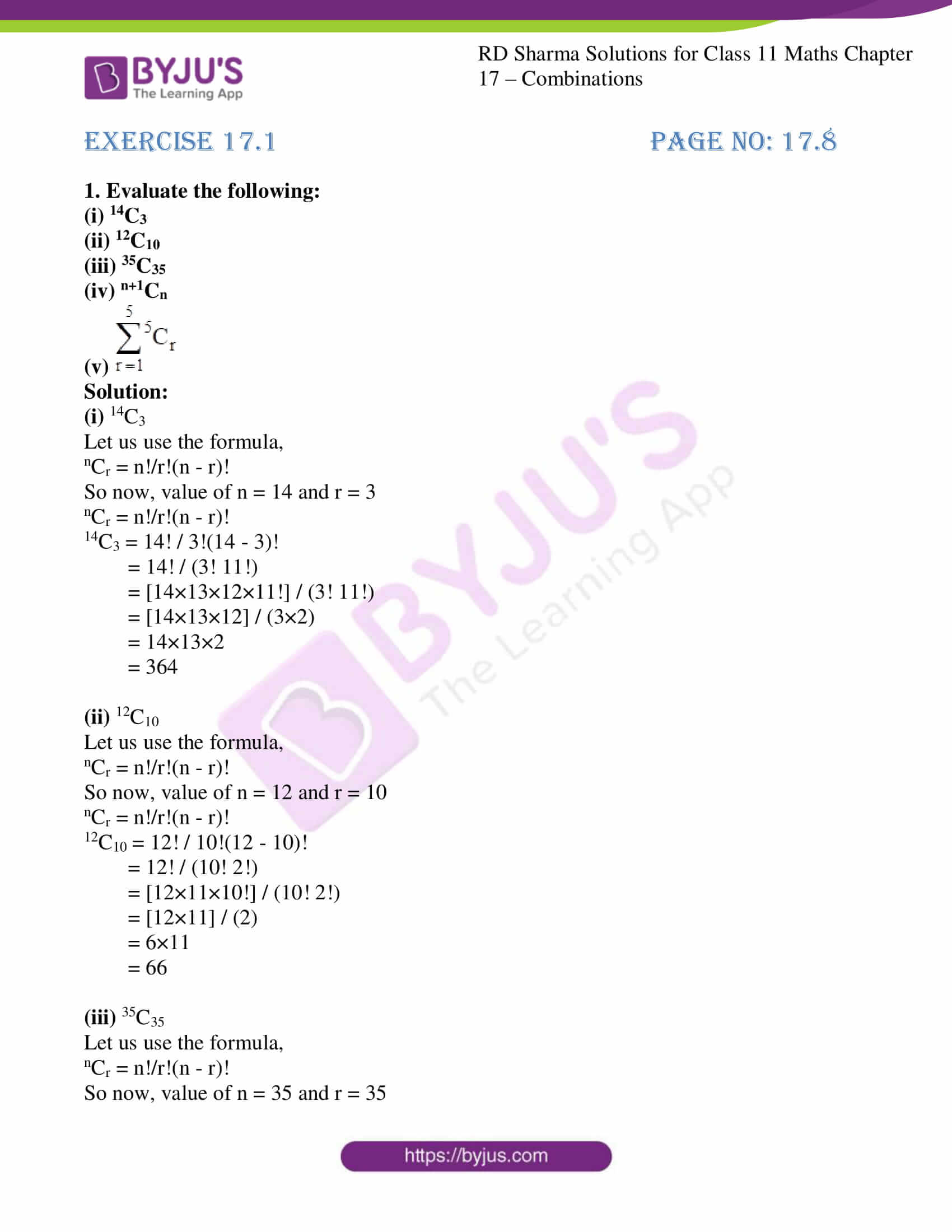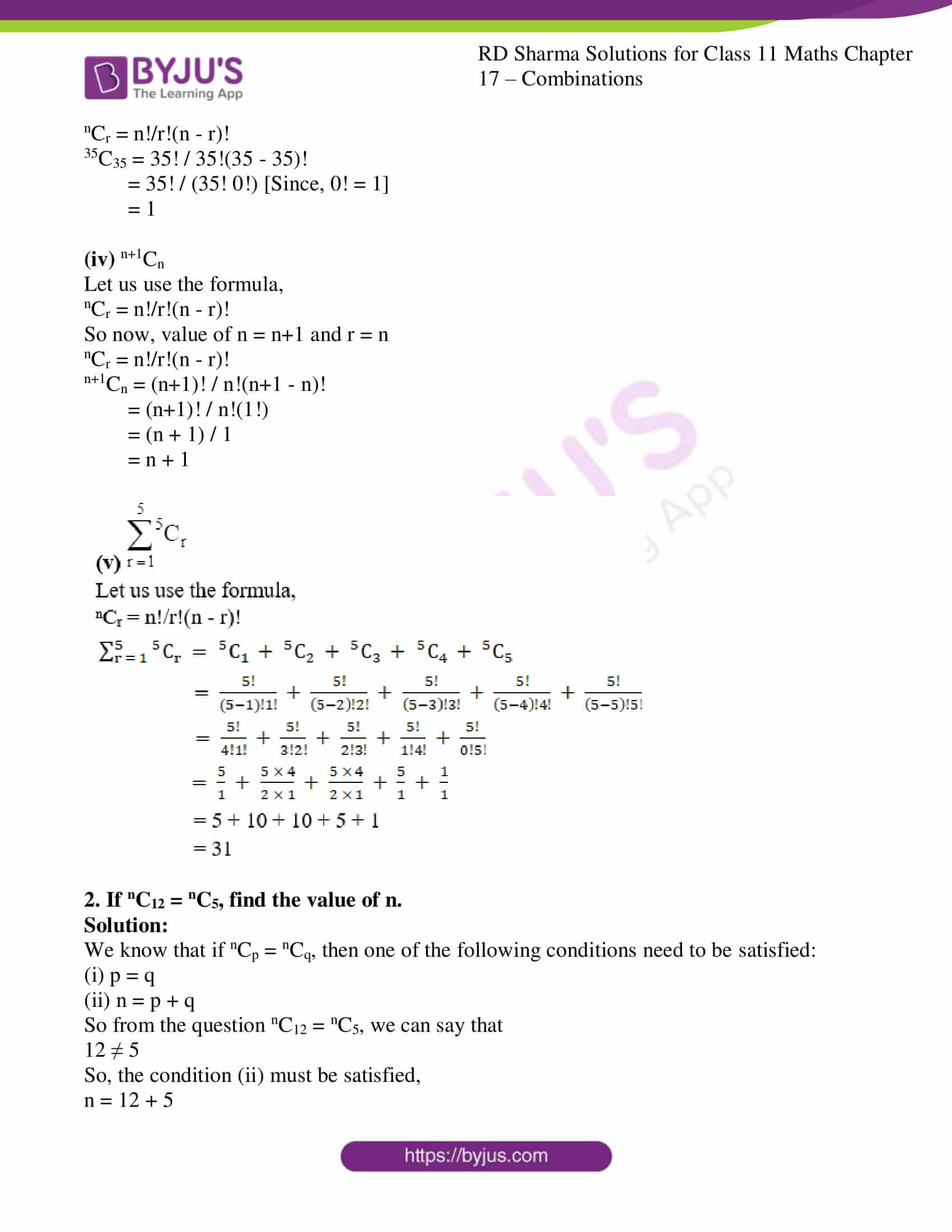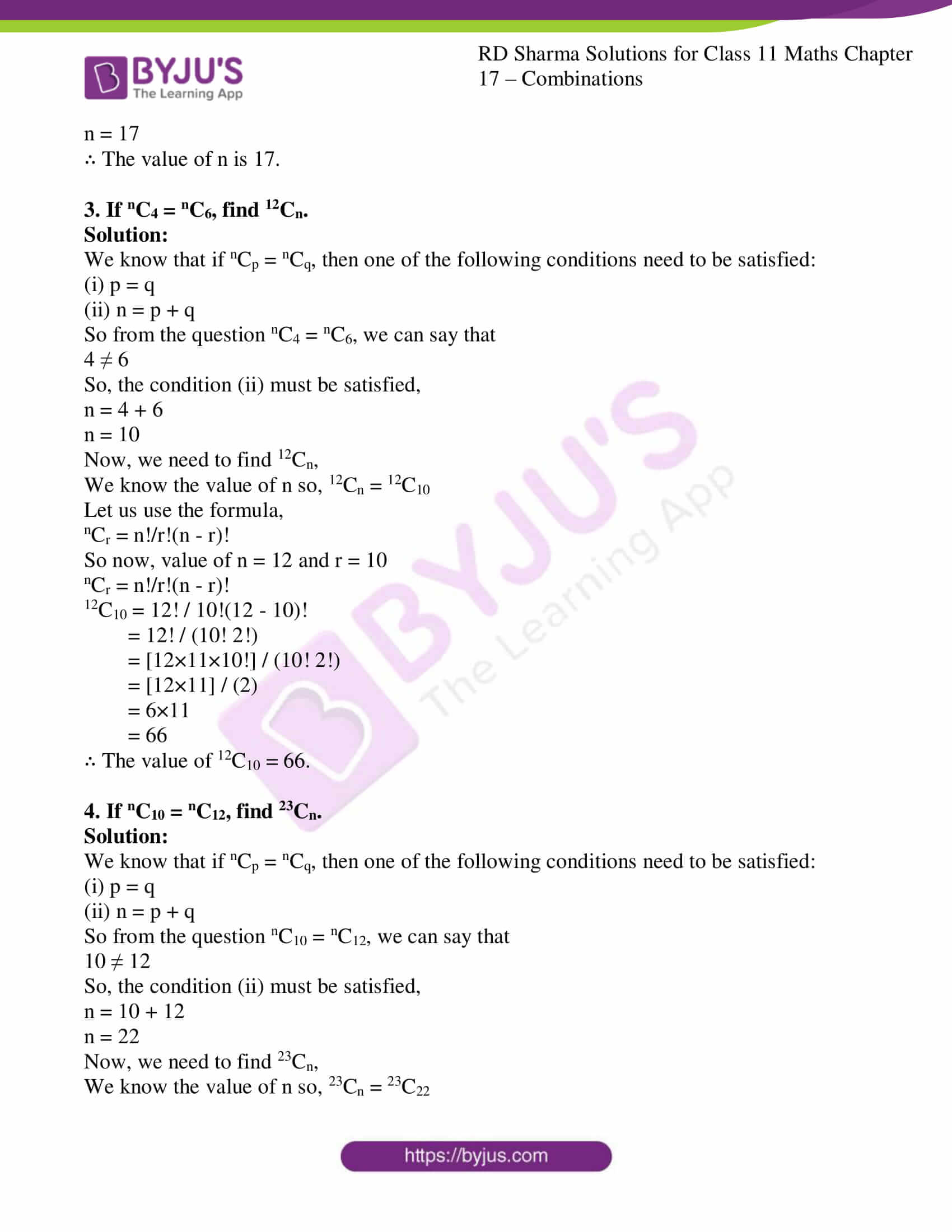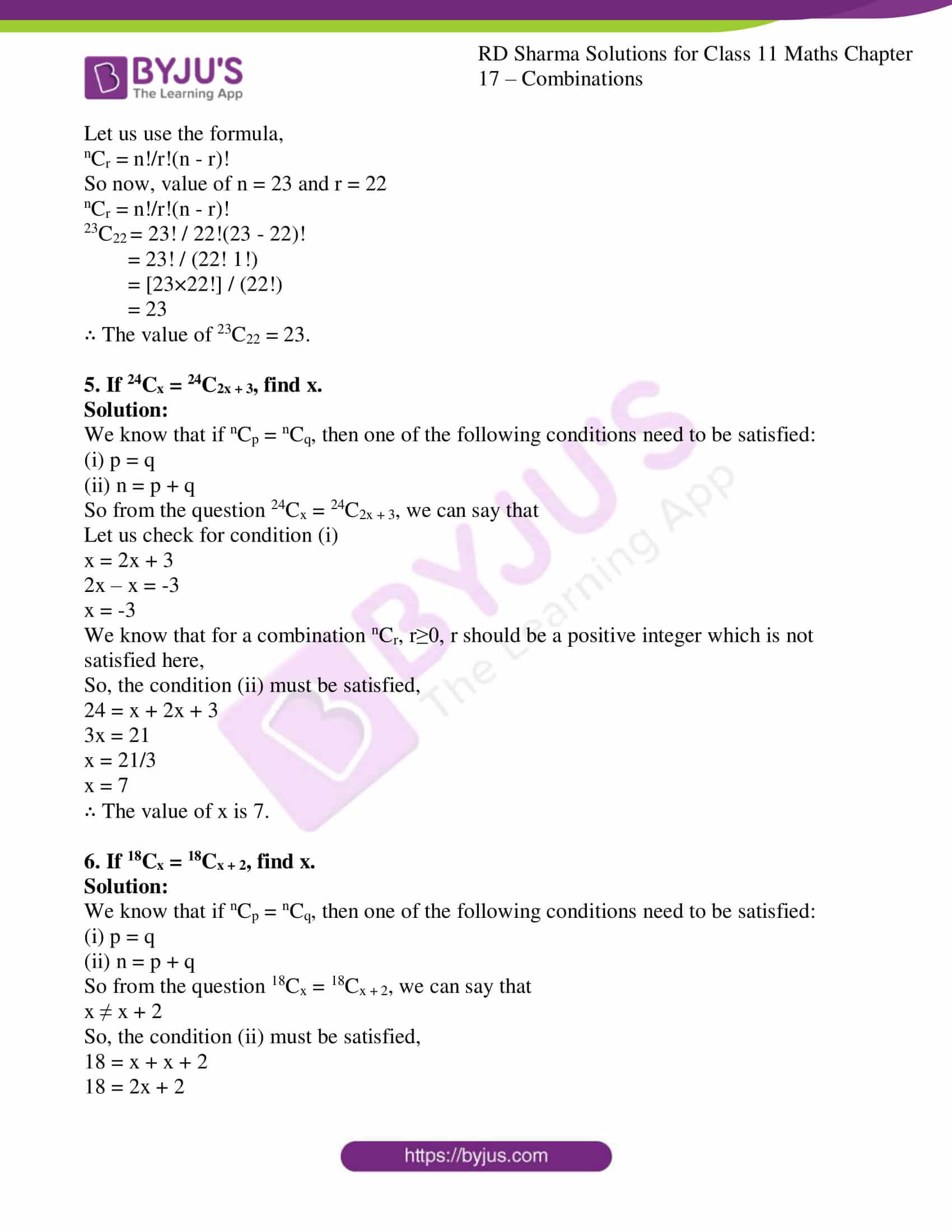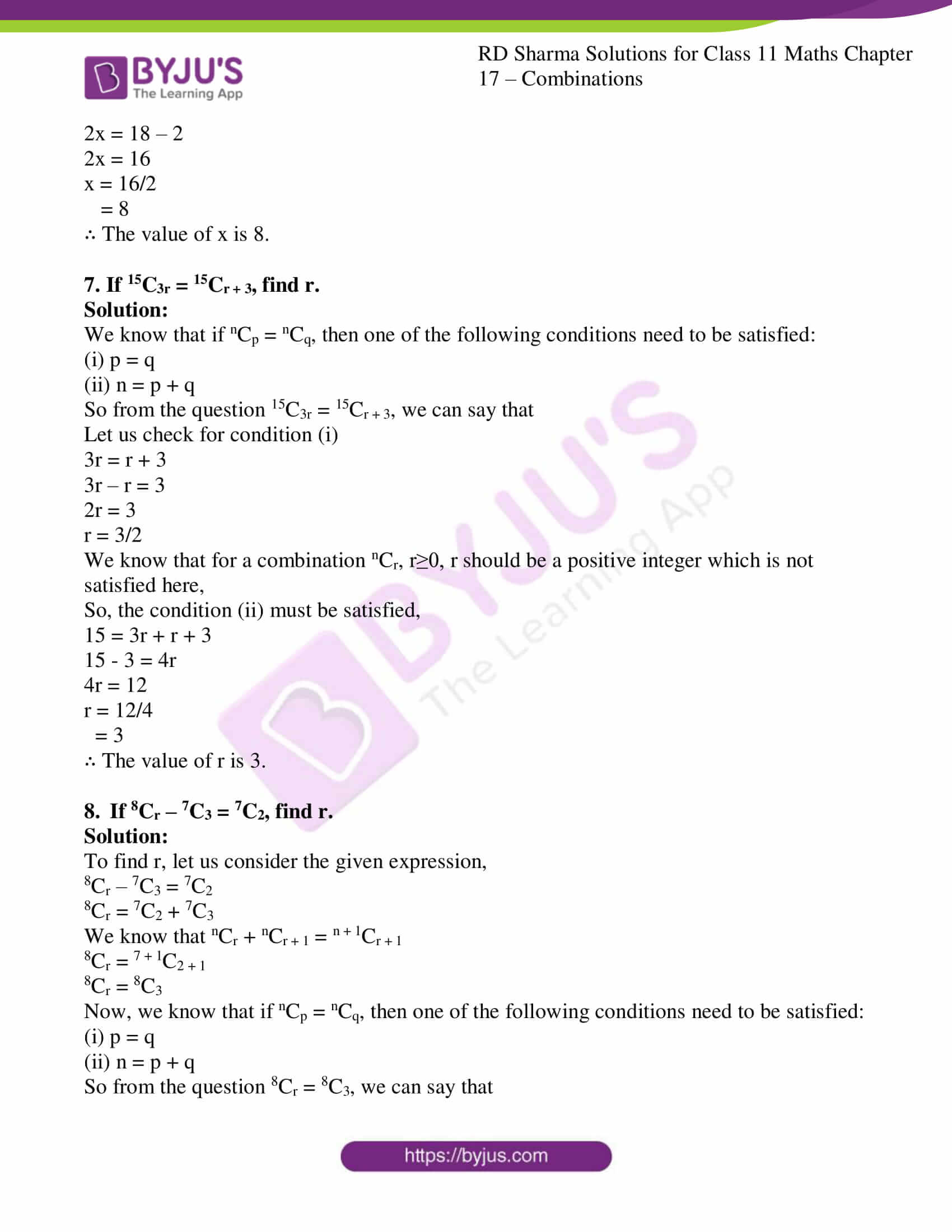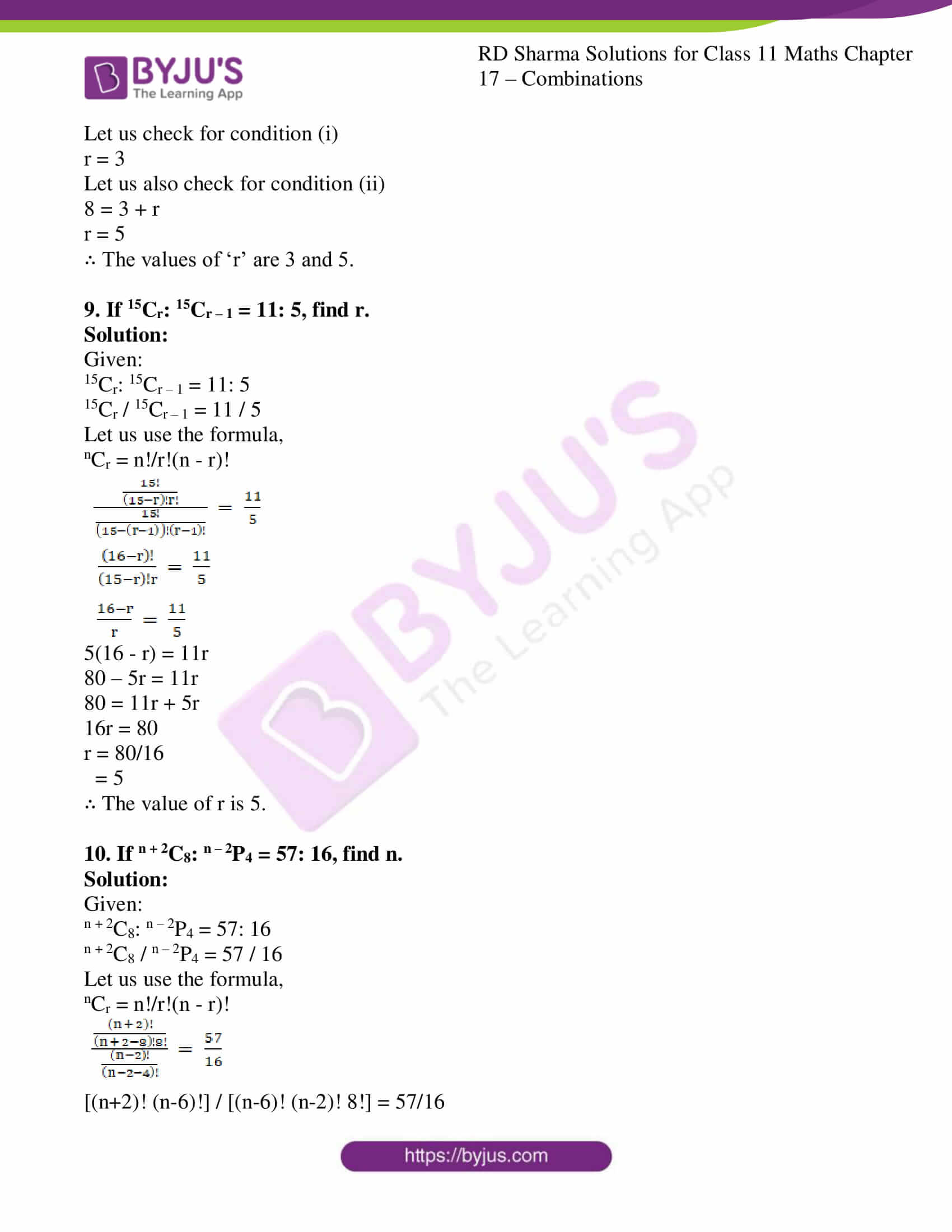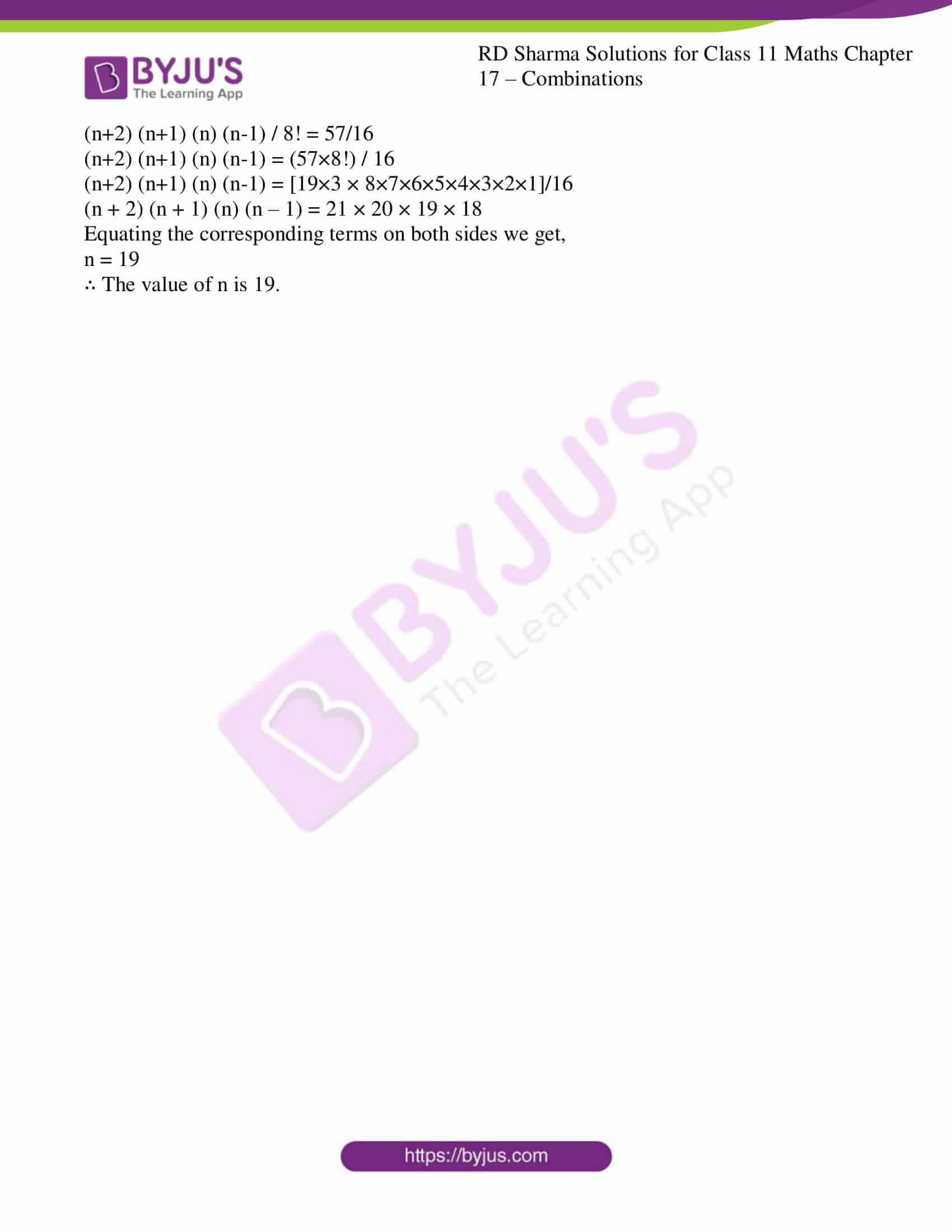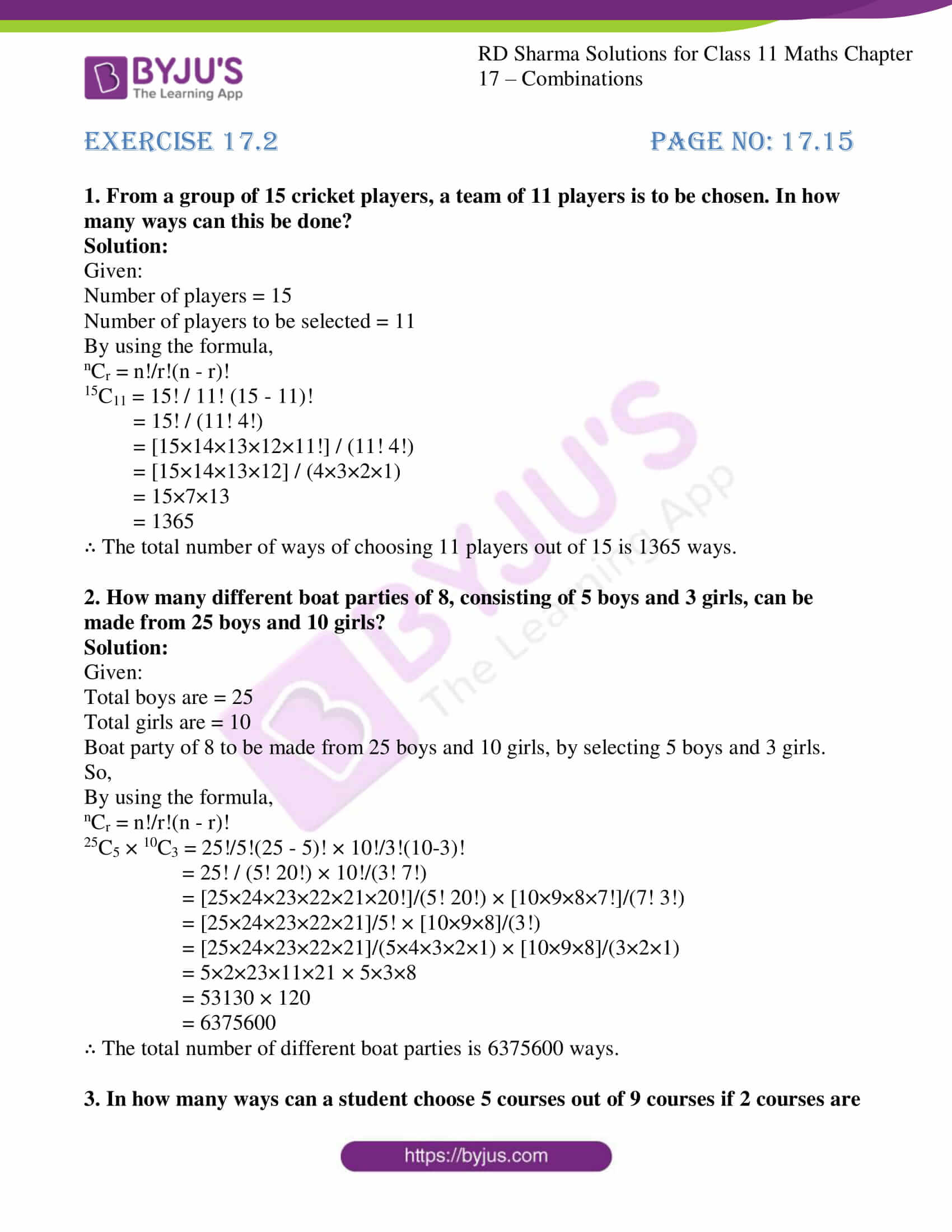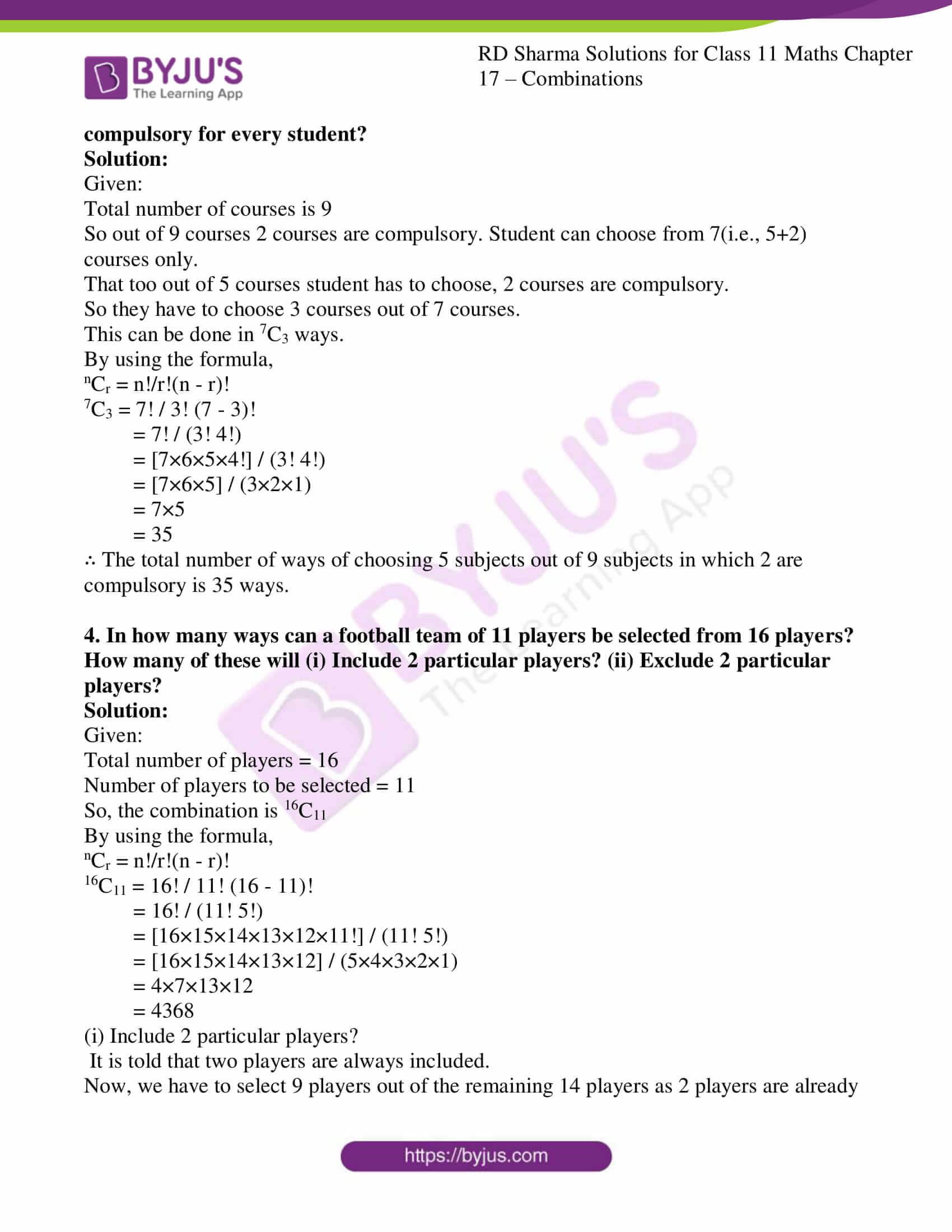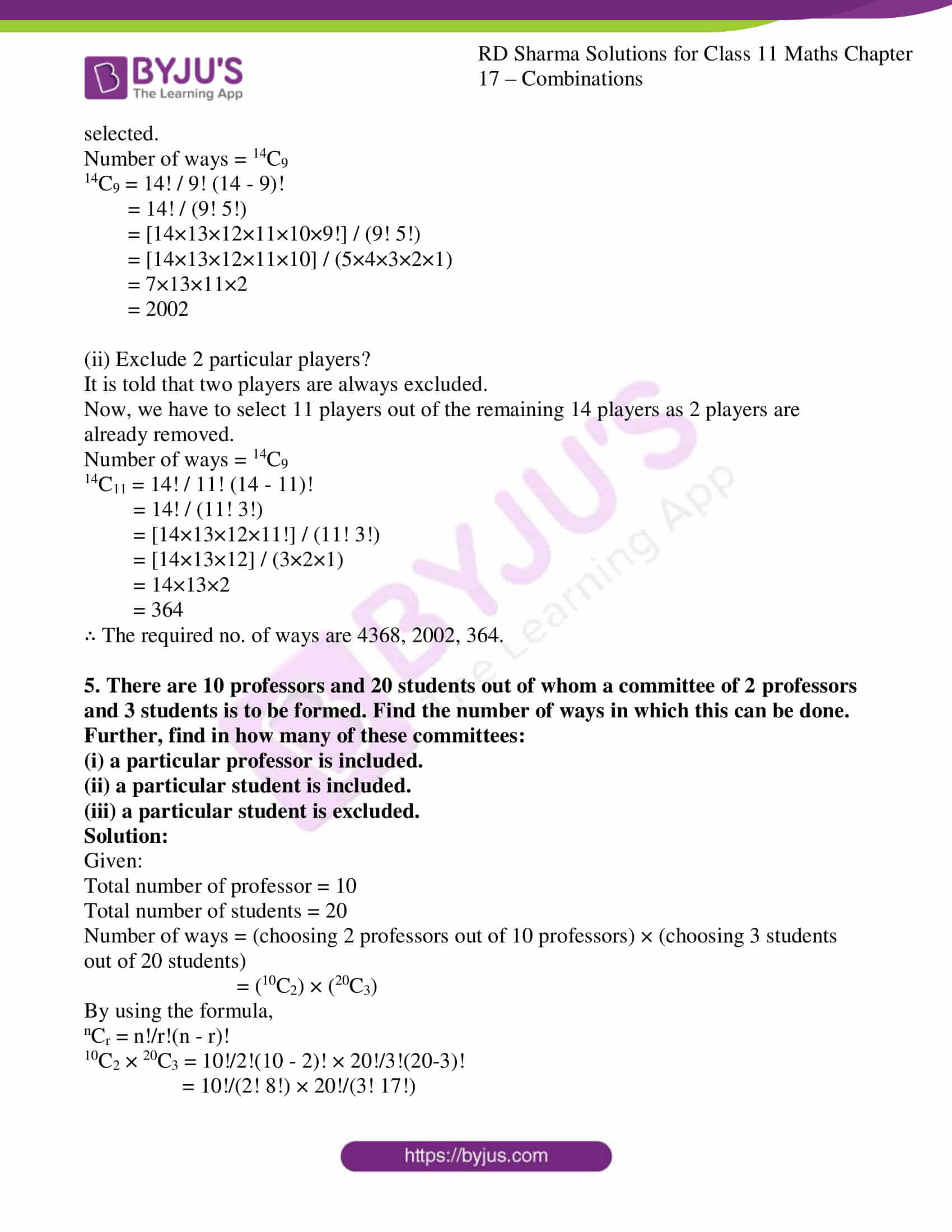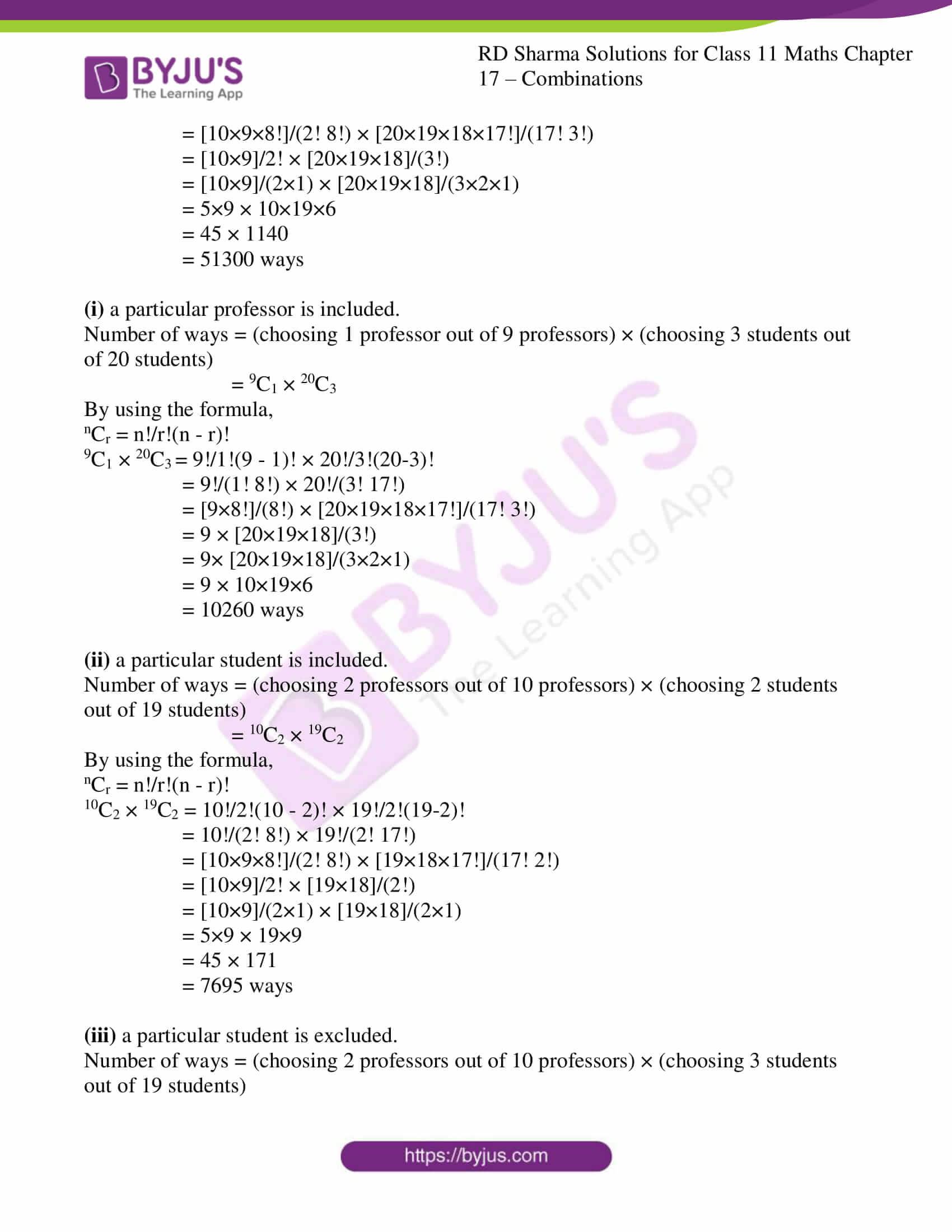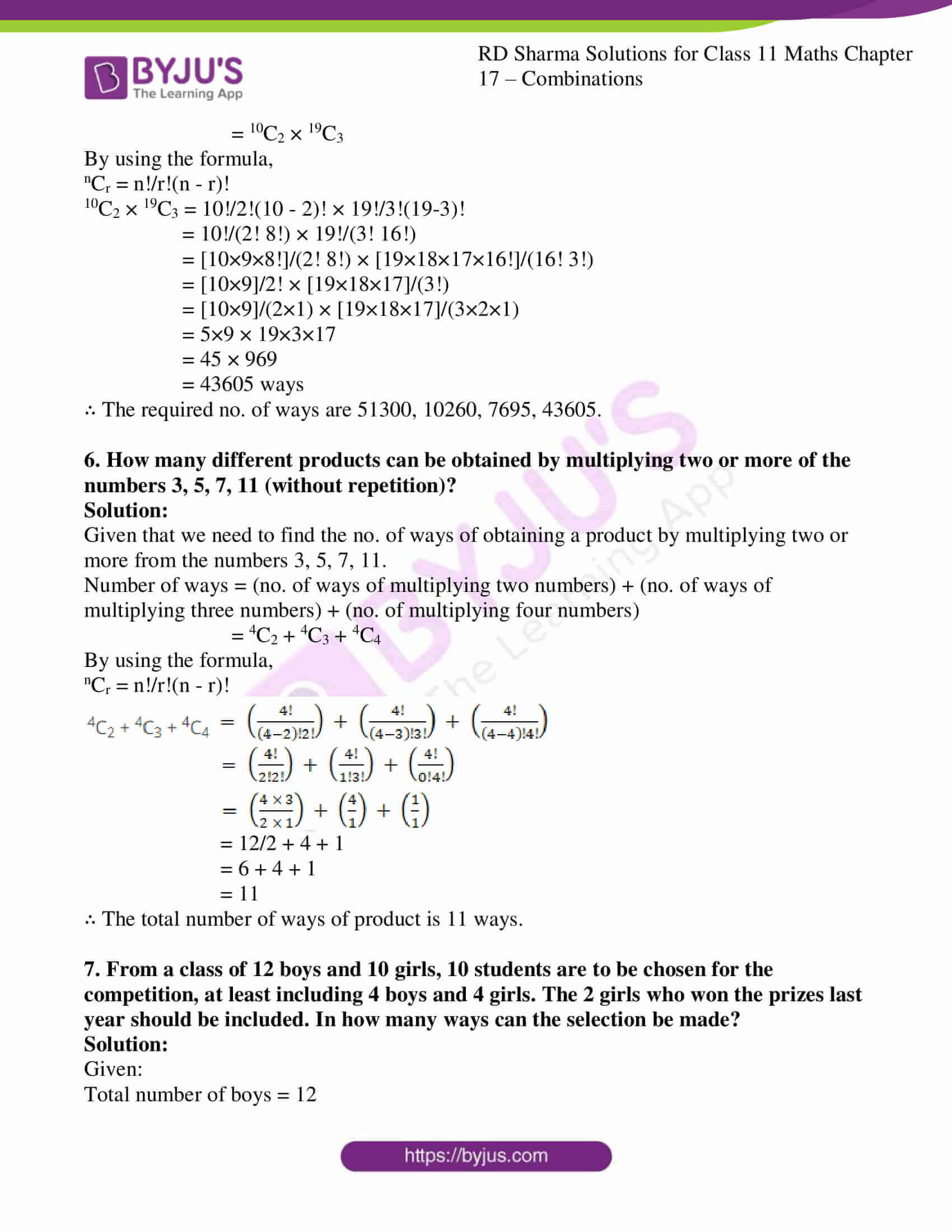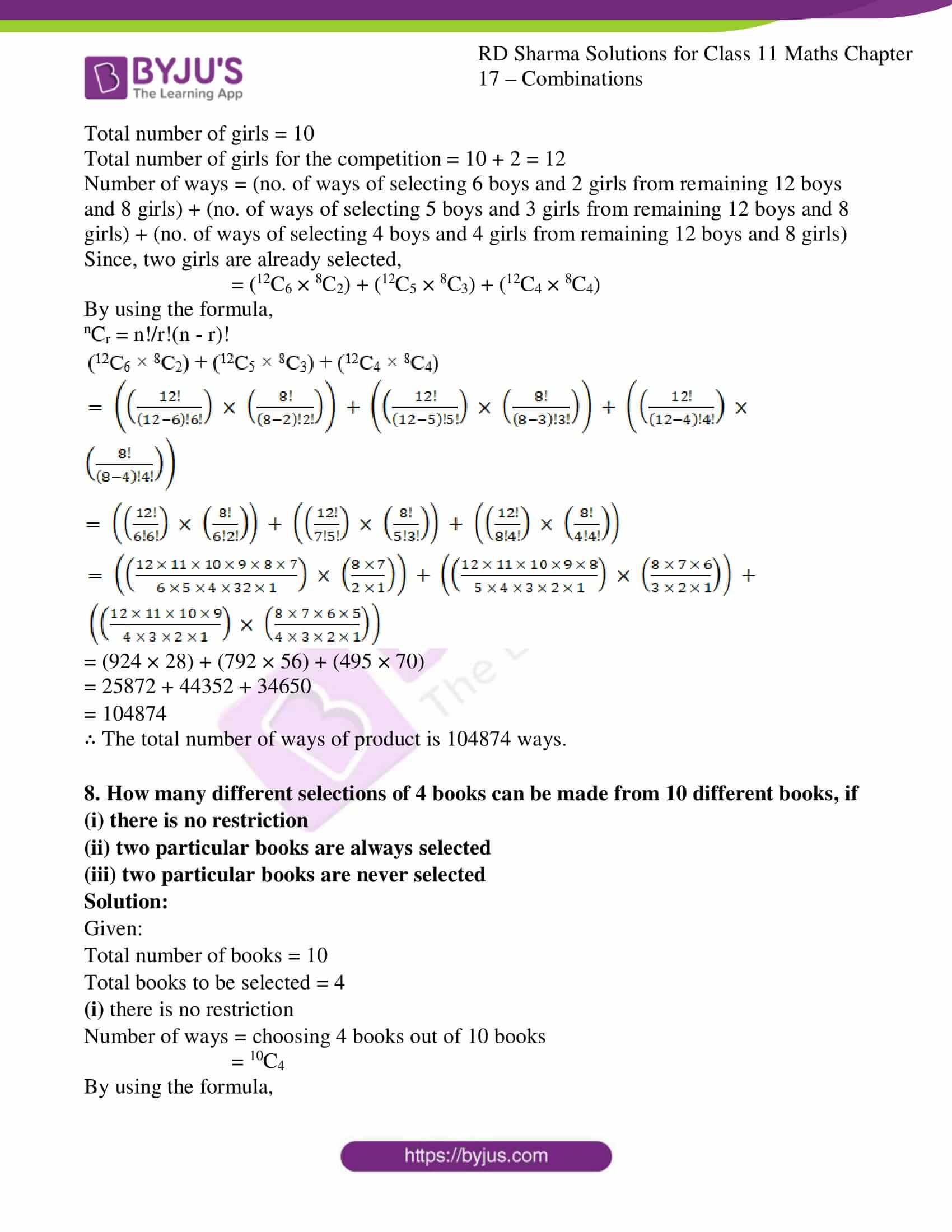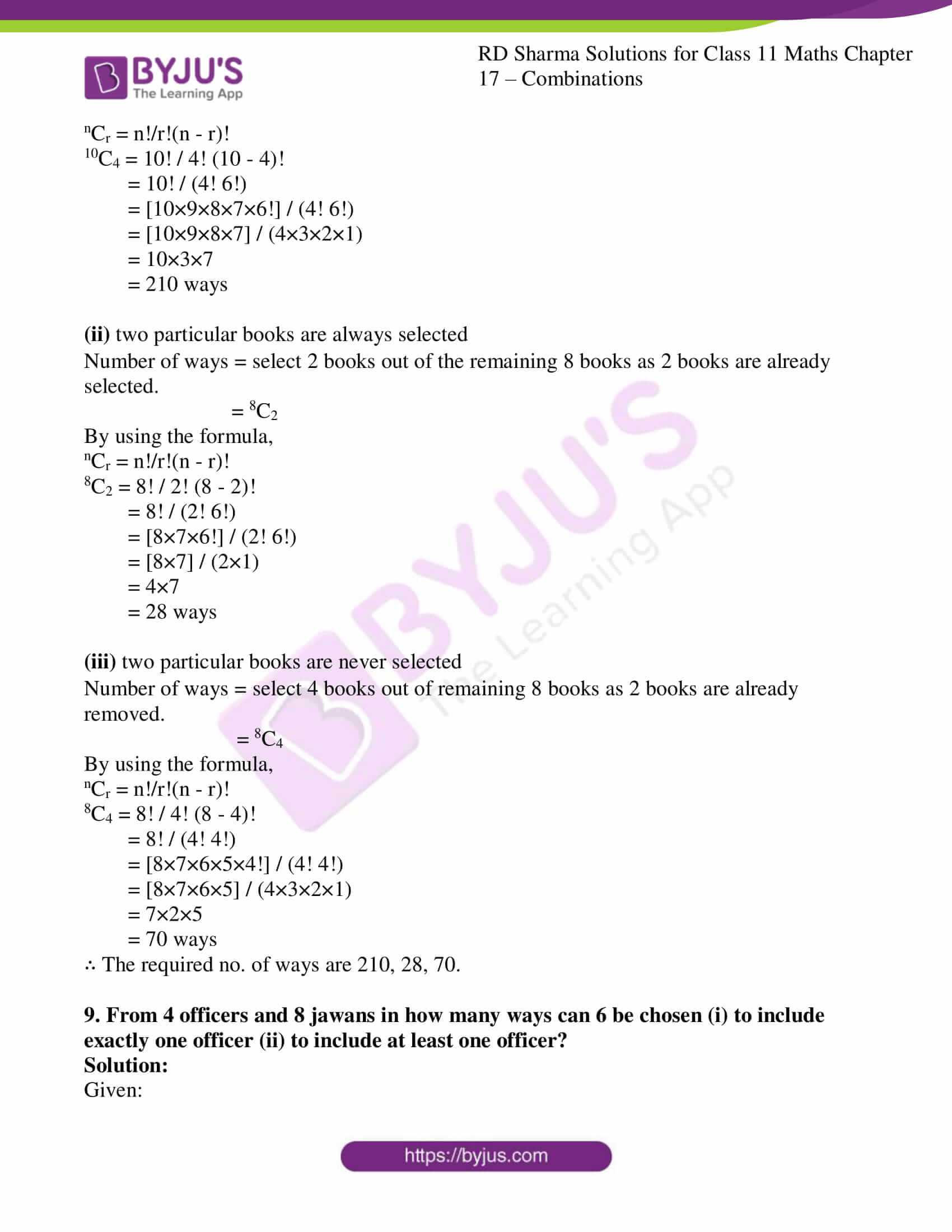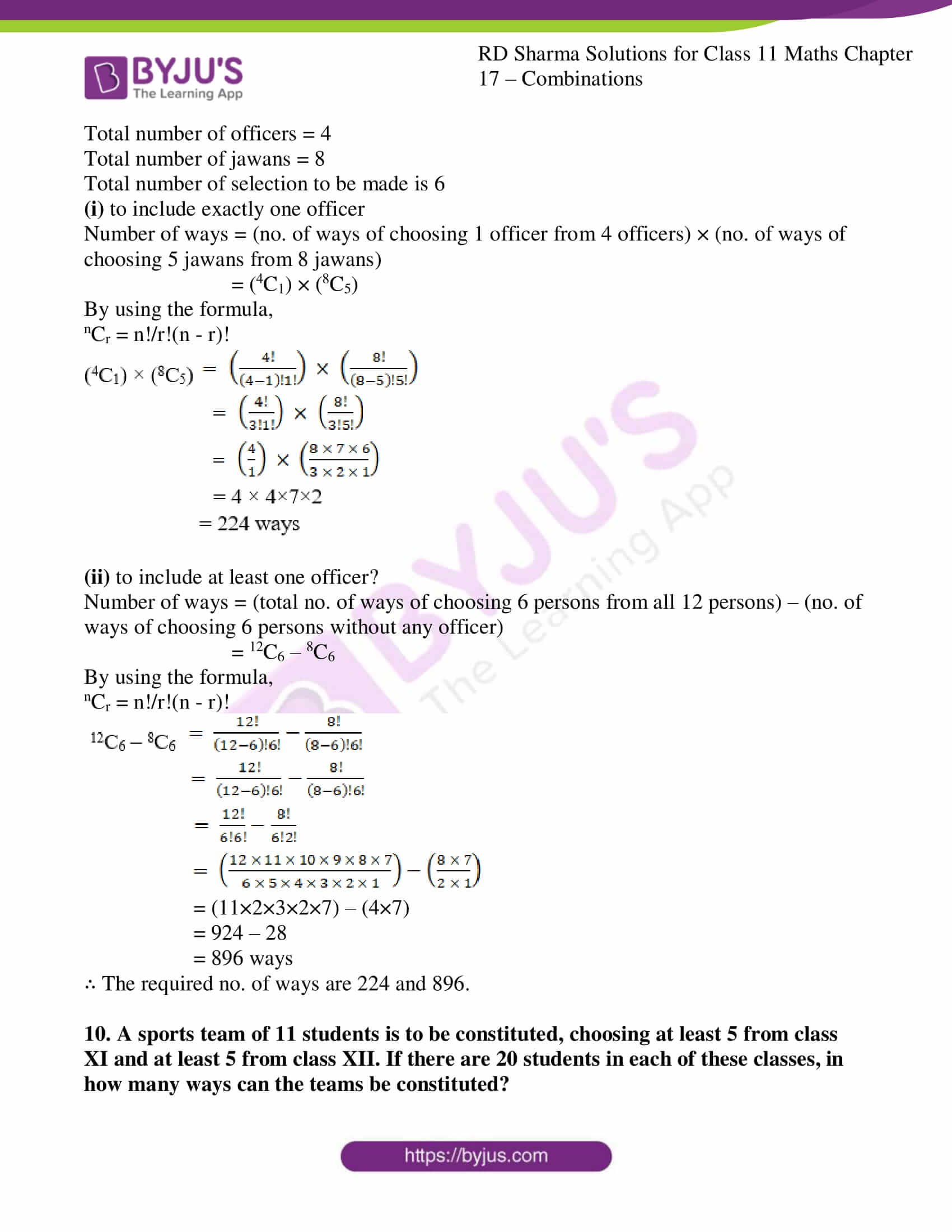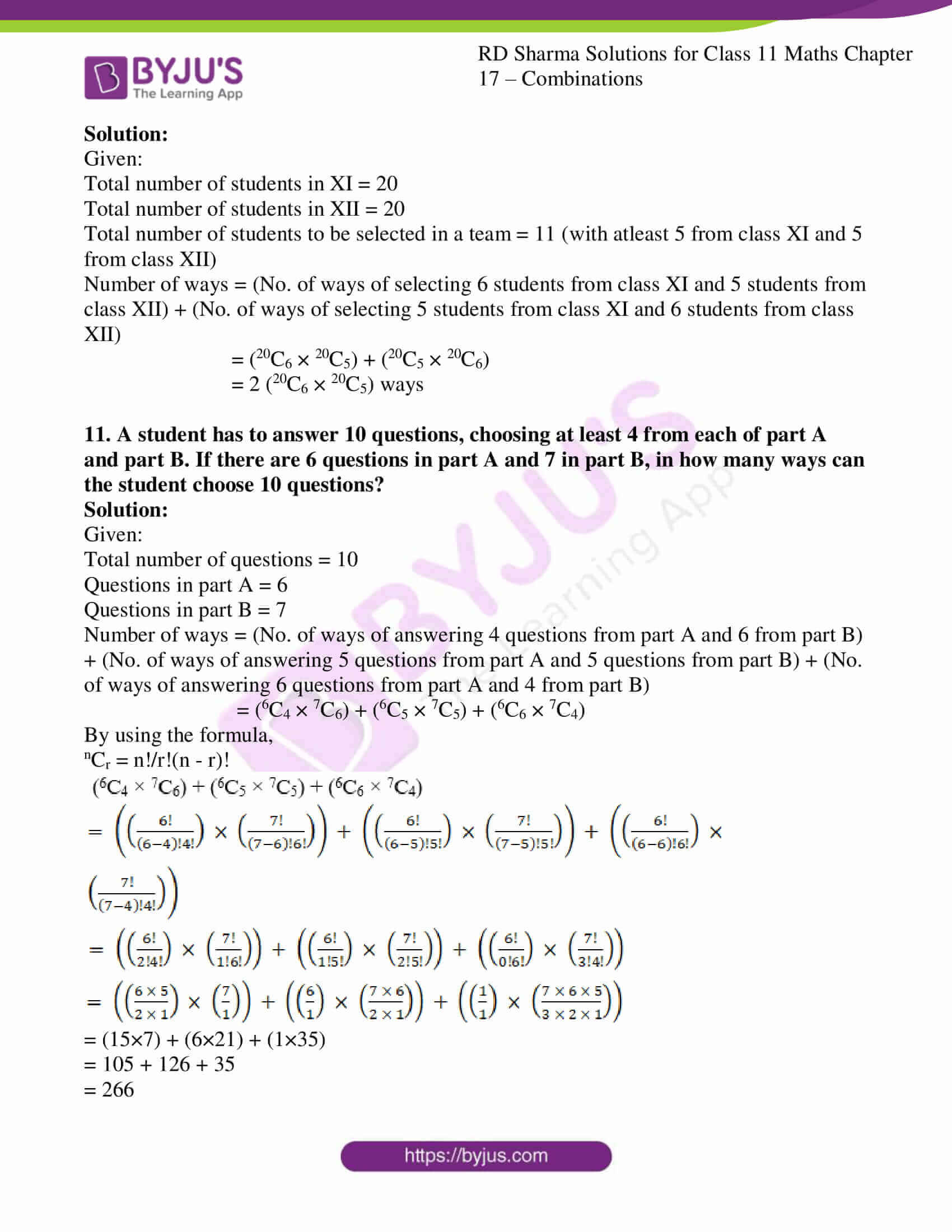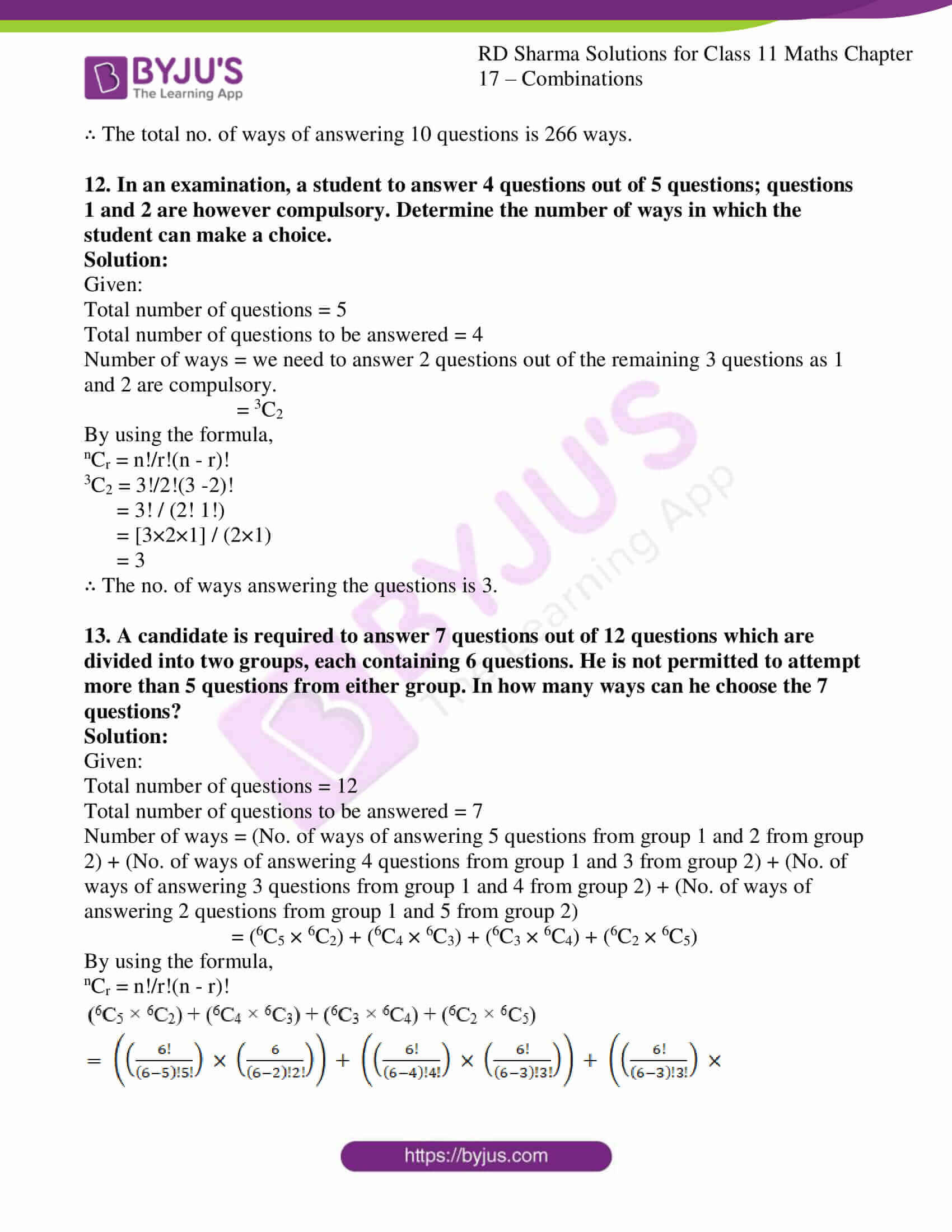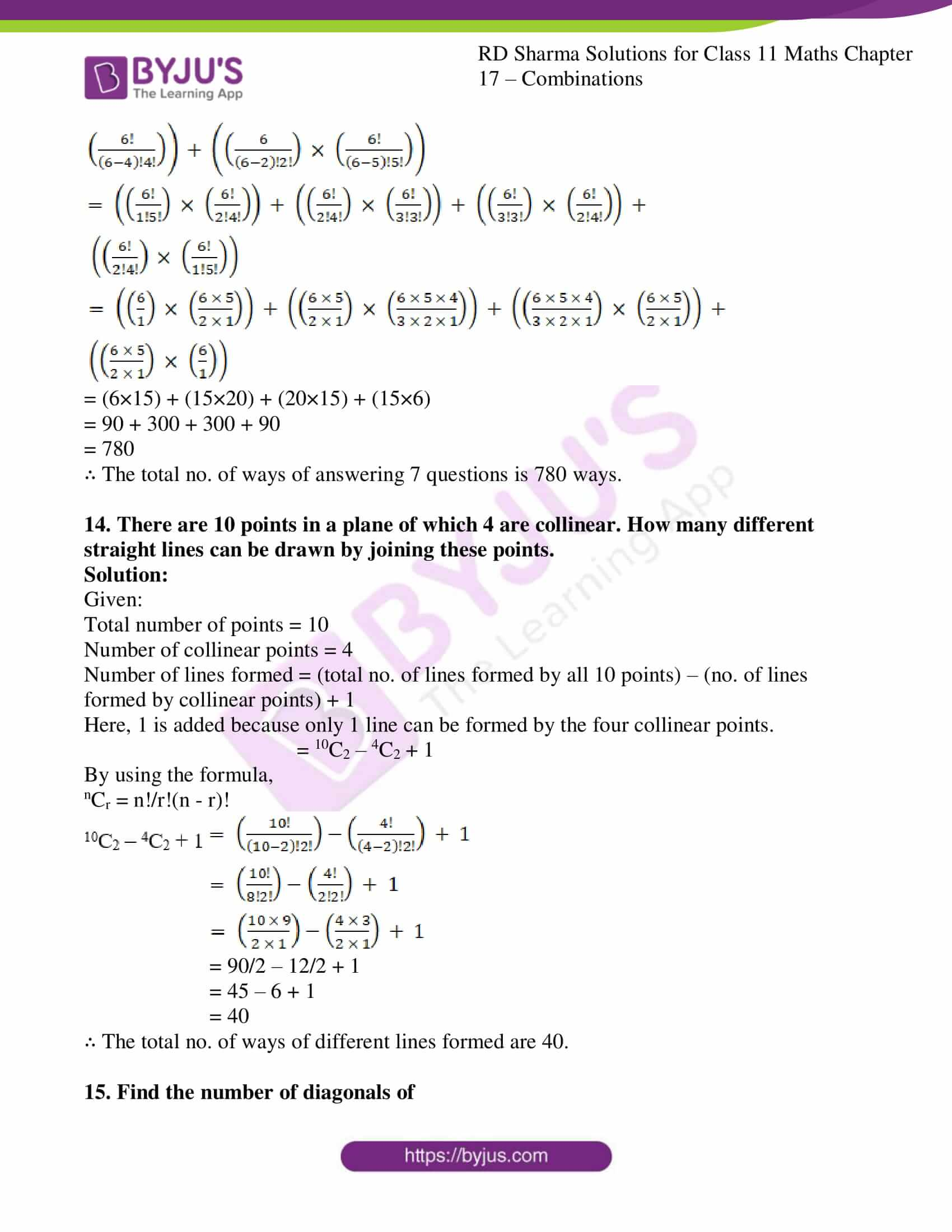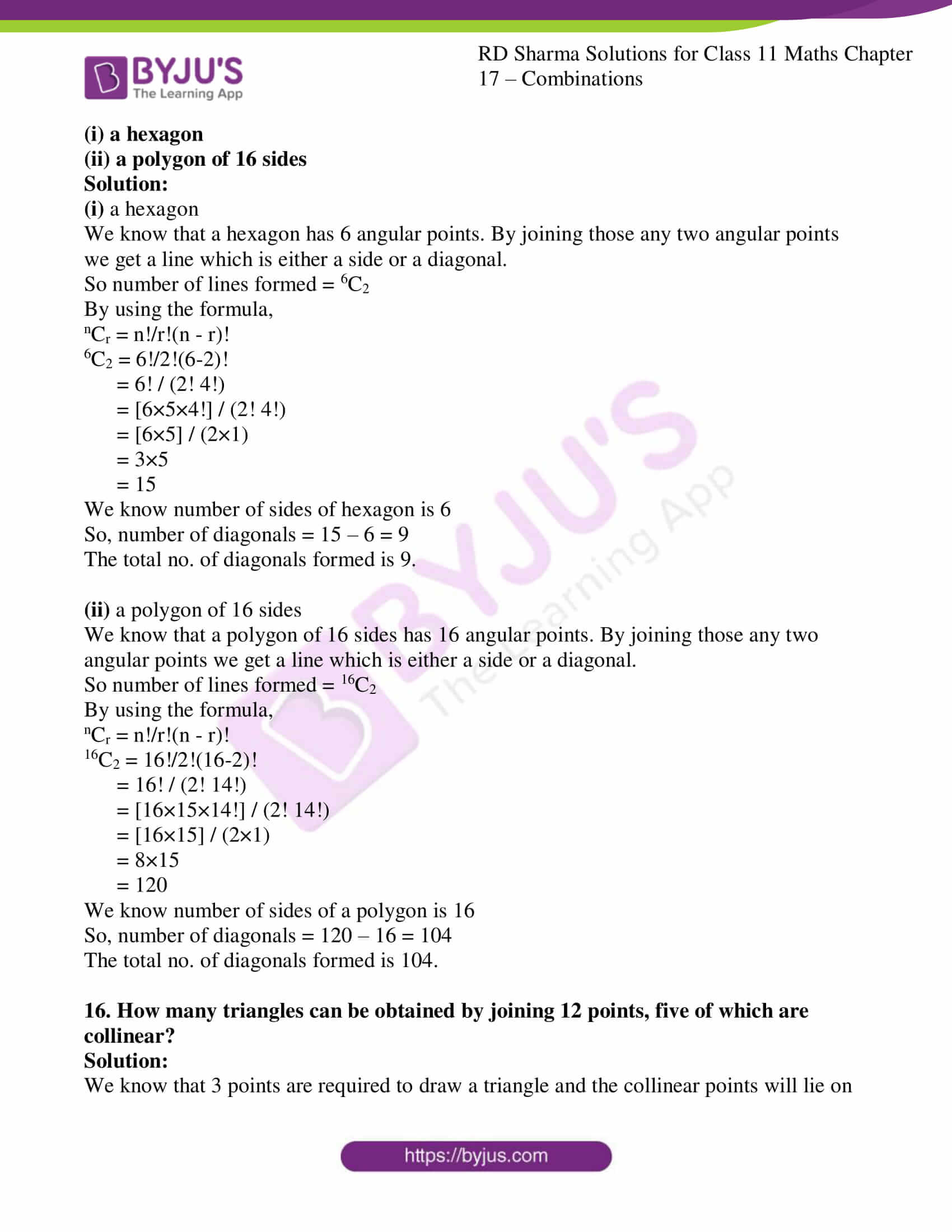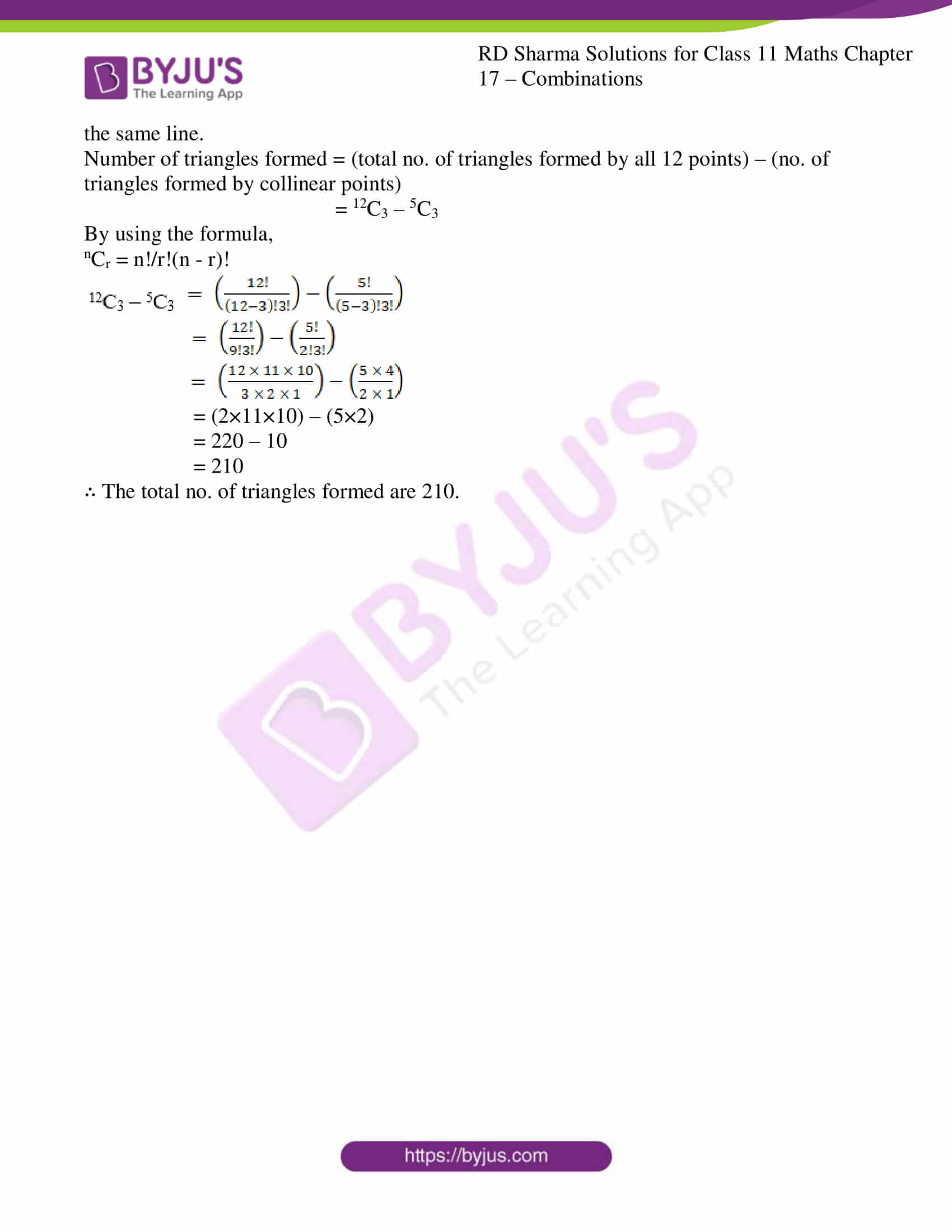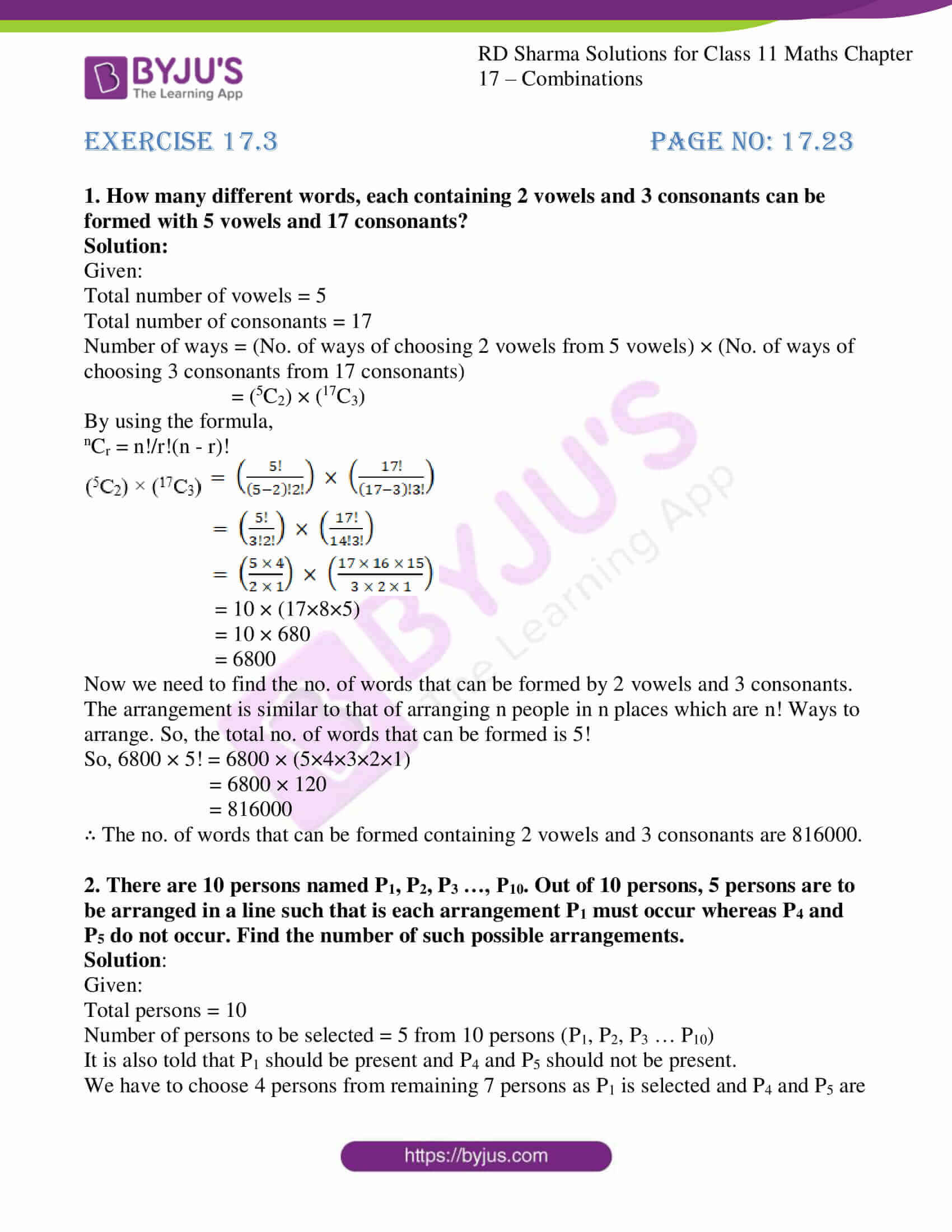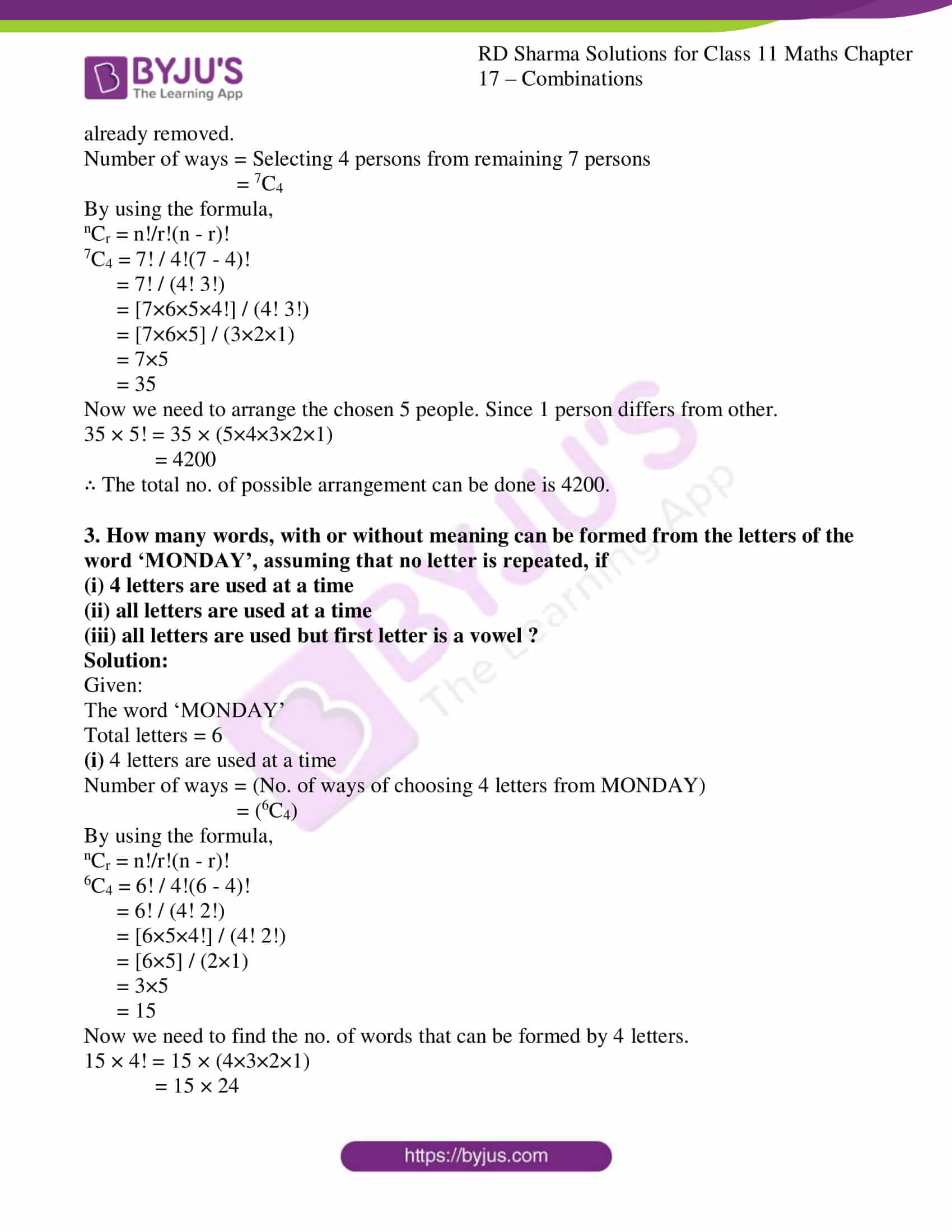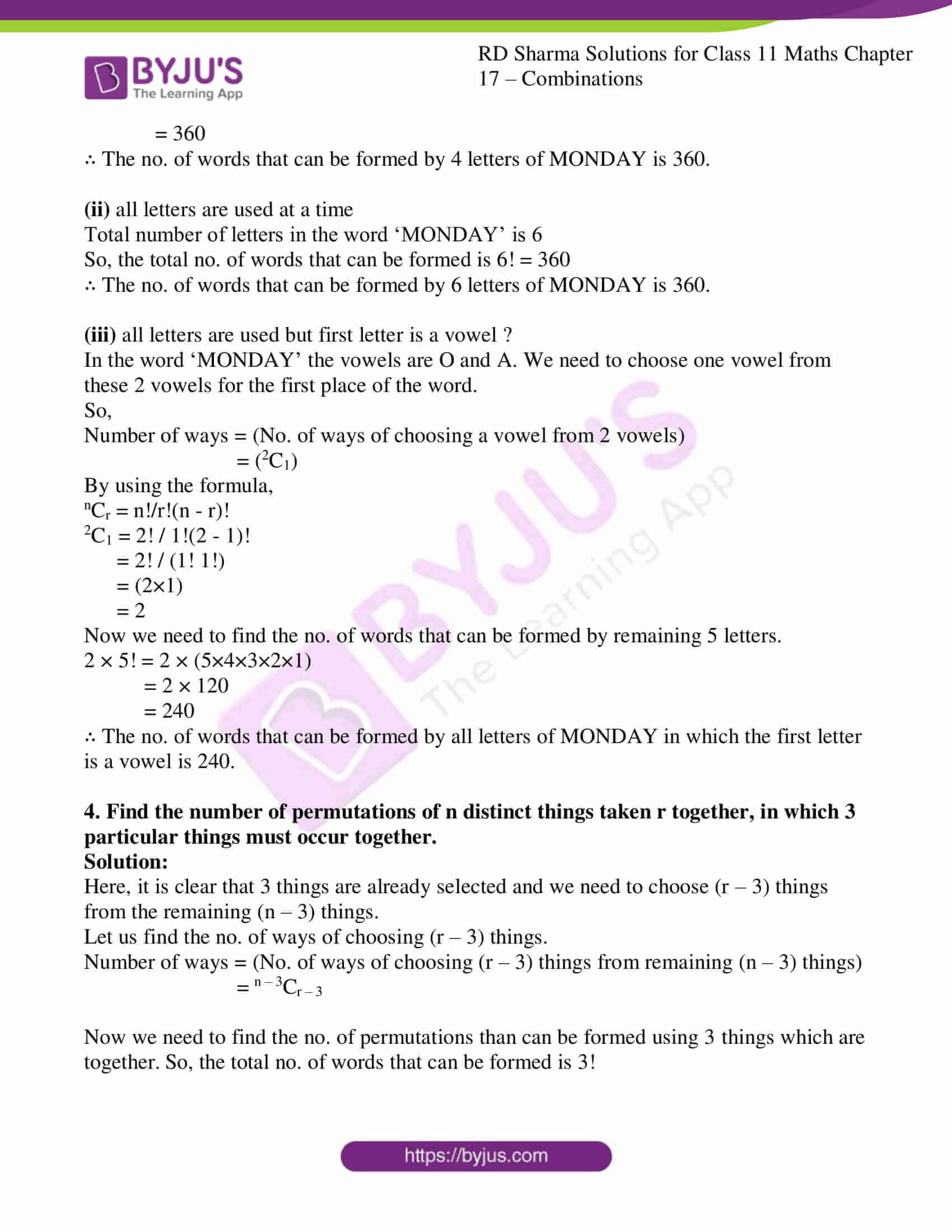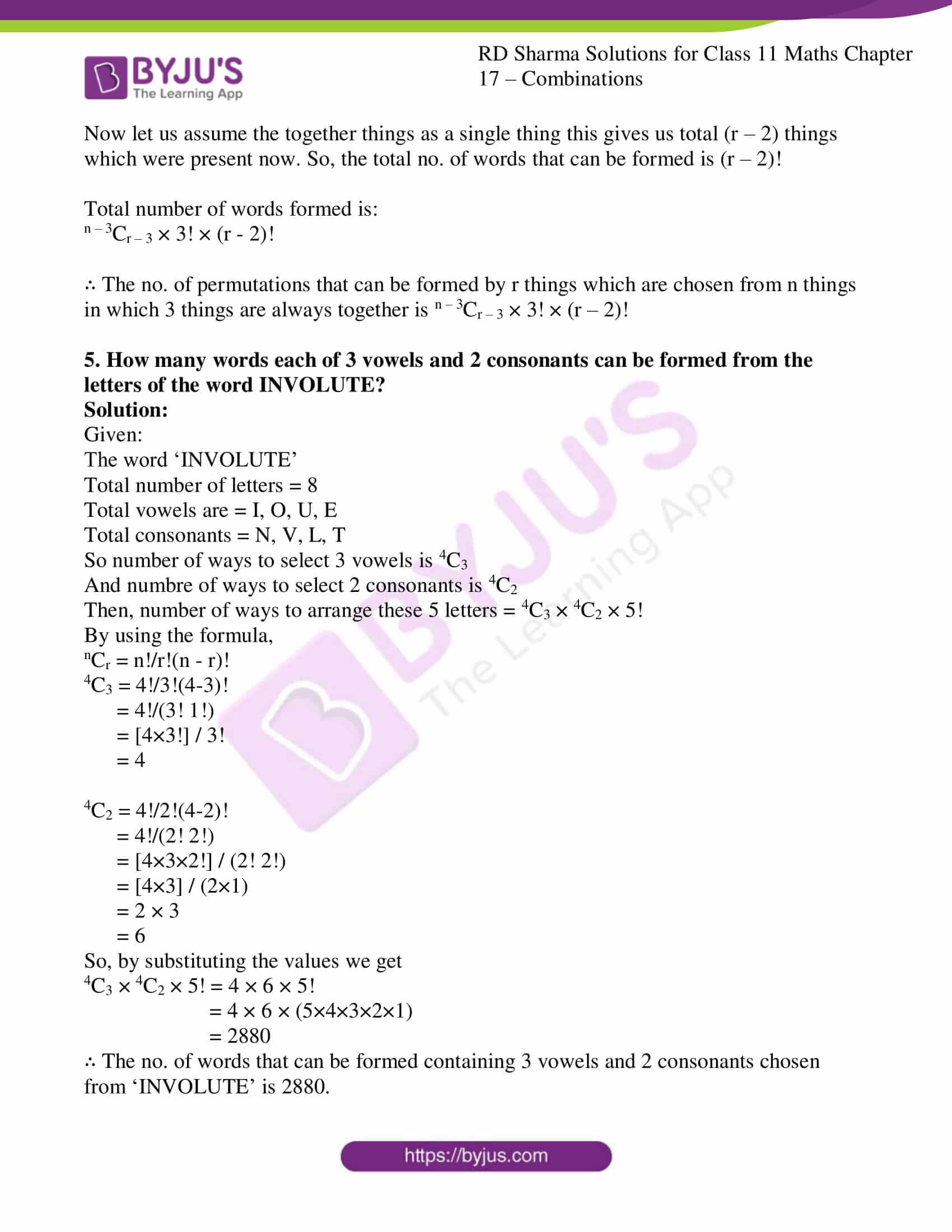### Access answers to RD Sharma Solutions for Class 11 Maths Chapter 17 – Combinations

EXERCISE 17.1 PAGE NO: 17.8

1. Evaluate the following:

(i) 14C3

(ii) 12C10

(iii) 35C35

(iv) n+1Cn

(v)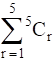Solution:

(i) 14C3

Let us use the formula,

nCr = n!/r!(n – r)!

So now, value of n = 14 and r = 3

nCr = n!/r!(n – r)!

14C3 = 14! / 3!(14 – 3)!

= 14! / (3! 11!)

= [14×13×12×11!] / (3! 11!)

= [14×13×12] / (3×2)

= 14×13×2

= 364

(ii) 12C10

Let us use the formula,

nCr = n!/r!(n – r)!

So now, value of n = 12 and r = 10

nCr = n!/r!(n – r)!

12C10 = 12! / 10!(12 – 10)!

= 12! / (10! 2!)

= [12×11×10!] / (10! 2!)

= [12×11] / (2)

= 6×11

= 66

(iii) 35C35

Let us use the formula,

nCr = n!/r!(n – r)!

So now, value of n = 35 and r = 35

nCr = n!/r!(n – r)!

35C35 = 35! / 35!(35 – 35)!

= 35! / (35! 0!) [Since, 0! = 1]

= 1

(iv) n+1Cn

Let us use the formula,

nCr = n!/r!(n – r)!

So now, value of n = n+1 and r = n

nCr = n!/r!(n – r)!

n+1Cn = (n+1)! / n!(n+1 – n)!

= (n+1)! / n!(1!)

= (n + 1) / 1

= n + 1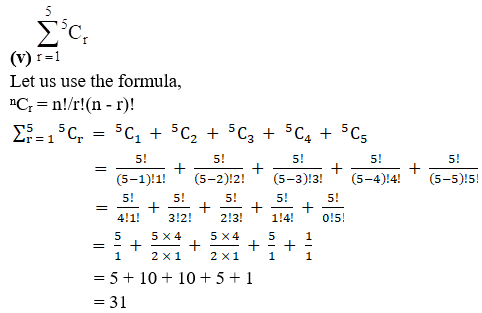2. If nC12 = nC5, find the value of n.

Solution:

We know that if nCp = nCq, then one of the following conditions need to be satisfied:

(i) p = q

(ii) n = p + q

So from the question nC12 = nC5, we can say that

12 ≠ 5

So, the condition (ii) must be satisfied,

n = 12 + 5

n = 17

∴ The value of n is 17.

3. If nC4 = nC6, find 12Cn.

Solution:

We know that if nCp = nCq, then one of the following conditions need to be satisfied:

(i) p = q

(ii) n = p + q

So from the question nC4 = nC6, we can say that

4 ≠ 6

So, the condition (ii) must be satisfied,

n = 4 + 6

n = 10

Now, we need to find 12Cn,

We know the value of n so, 12Cn = 12C10

Let us use the formula,

nCr = n!/r!(n – r)!

So now, value of n = 12 and r = 10

nCr = n!/r!(n – r)!

12C10 = 12! / 10!(12 – 10)!

= 12! / (10! 2!)

= [12×11×10!] / (10! 2!)

= [12×11] / (2)

= 6×11

= 66

∴ The value of 12C10 = 66.

4. If nC10 = nC12, find 23Cn.

Solution:

We know that if nCp = nCq, then one of the following conditions need to be satisfied:

(i) p = q

(ii) n = p + q

So from the question nC10 = nC12, we can say that

10 ≠ 12

So, the condition (ii) must be satisfied,

n = 10 + 12

n = 22

Now, we need to find 23Cn,

We know the value of n so, 23Cn = 23C22

Let us use the formula,

nCr = n!/r!(n – r)!

So now, value of n = 23 and r = 22

nCr = n!/r!(n – r)!

23C22 = 23! / 22!(23 – 22)!

= 23! / (22! 1!)

= [23×22!] / (22!)

= 23

∴ The value of 23C22 = 23.

5. If 24Cx = 24C2x + 3, find x.

Solution:

We know that if nCp = nCq, then one of the following conditions need to be satisfied:

(i) p = q

(ii) n = p + q

So from the question 24Cx = 24C2x + 3, we can say that

Let us check for condition (i)

x = 2x + 3

2x – x = -3

x = -3

We know that for a combination nCr, r≥0, r should be a positive integer which is not satisfied here,

So, the condition (ii) must be satisfied,

24 = x + 2x + 3

3x = 21

x = 21/3

x = 7

∴ The value of x is 7.

6. If 18Cx = 18Cx + 2, find x.

Solution:

We know that if nCp = nCq, then one of the following conditions need to be satisfied:

(i) p = q

(ii) n = p + q

So from the question 18Cx = 18Cx + 2, we can say that

x ≠ x + 2

So, the condition (ii) must be satisfied,

18 = x + x + 2

18 = 2x + 2

2x = 18 – 2

2x = 16

x = 16/2

= 8

∴ The value of x is 8.

7. If 15C3r = 15Cr + 3, find r.

Solution:

We know that if nCp = nCq, then one of the following conditions need to be satisfied:

(i) p = q

(ii) n = p + q

So from the question 15C3r = 15Cr + 3, we can say that

Let us check for condition (i)

3r = r + 3

3r – r = 3

2r = 3

r = 3/2

We know that for a combination nCr, r≥0, r should be a positive integer which is not satisfied here,

So, the condition (ii) must be satisfied,

15 = 3r + r + 3

15 – 3 = 4r

4r = 12

r = 12/4

= 3

∴ The value of r is 3.

8. If 8Cr – 7C3 = 7C2, find r.

Solution:

To find r, let us consider the given expression,

8Cr – 7C3 = 7C2

8Cr = 7C2 + 7C3

We know that nCr + nCr + 1 = n + 1Cr + 1

8Cr = 7 + 1C2 + 1

8Cr = 8C3

Now, we know that if nCp = nCq, then one of the following conditions need to be satisfied:

(i) p = q

(ii) n = p + q

So from the question 8Cr = 8C3, we can say that

Let us check for condition (i)

r = 3

Let us also check for condition (ii)

8 = 3 + r

r = 5

∴ The values of ‘r’ are 3 and 5.

9. If 15Cr15Cr – 1 = 11: 5, find r.

Solution:

Given:

15Cr15Cr – 1 = 11: 5

15Cr15Cr – 1 = 11 / 5

Let us use the formula,

nCr = n!/r!(n – r)!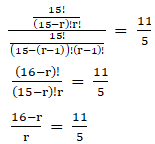5(16 – r) = 11r

80 – 5r = 11r

80 = 11r + 5r

16r = 80

r = 80/16

= 5

∴ The value of r is 5.

10. If n + 2C8n – 2P4 = 57: 16, find n.

Solution:

Given:

n + 2C8n – 2P4 = 57: 16

n + 2C8n – 2P4 = 57 / 16

Let us use the formula,

nCr = n!/r!(n – r)!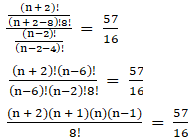[(n+2)! (n-6)!] / [(n-6)! (n-2)! 8!] = 57/16

(n+2) (n+1) (n) (n-1) / 8! = 57/16

(n+2) (n+1) (n) (n-1) = (57×8!) / 16

(n+2) (n+1) (n) (n-1) = [19×3 × 8×7×6×5×4×3×2×1]/16

(n + 2) (n + 1) (n) (n – 1) = 21 × 20 × 19 × 18

Equating the corresponding terms on both sides we get,

n = 19

∴ The value of n is 19.

EXERCISE 17.2 PAGE NO: 17.15

1. From a group of 15 cricket players, a team of 11 players is to be chosen. In how many ways can this be done?

Solution:

Given:

Number of players = 15

Number of players to be selected = 11

By using the formula,

nCr = n!/r!(n – r)!

15C11 = 15! / 11! (15 – 11)!

= 15! / (11! 4!)

= [15×14×13×12×11!] / (11! 4!)

= [15×14×13×12] / (4×3×2×1)

= 15×7×13

= 1365

∴ The total number of ways of choosing 11 players out of 15 is 1365 ways.

2. How many different boat parties of 8, consisting of 5 boys and 3 girls, can be made from 25 boys and 10 girls?

Solution:

Given:

Total boys are = 25

Total girls are = 10

Boat party of 8 to be made from 25 boys and 10 girls, by selecting 5 boys and 3 girls.

So,

By using the formula,

nCr = n!/r!(n – r)!

25C5 × 10C3 = 25!/5!(25 – 5)! × 10!/3!(10-3)!

= 25! / (5! 20!) × 10!/(3! 7!)

= [25×24×23×22×21×20!]/(5! 20!) × [10×9×8×7!]/(7! 3!)

= [25×24×23×22×21]/5! × [10×9×8]/(3!)

= [25×24×23×22×21]/(5×4×3×2×1) × [10×9×8]/(3×2×1)

= 5×2×23×11×21 × 5×3×8

= 53130 × 120

= 6375600

∴ The total number of different boat parties is 6375600 ways.

3. In how many ways can a student choose 5 courses out of 9 courses if 2 courses are compulsory for every student?

Solution:

Given:

Total number of courses is 9

So out of 9 courses 2 courses are compulsory. Student can choose from 7(i.e., 5+2) courses only.

That too out of 5 courses student has to choose, 2 courses are compulsory.

So they have to choose 3 courses out of 7 courses.

This can be done in 7C3 ways.

By using the formula,

nCr = n!/r!(n – r)!

7C3 = 7! / 3! (7 – 3)!

= 7! / (3! 4!)

= [7×6×5×4!] / (3! 4!)

= [7×6×5] / (3×2×1)

= 7×5

= 35

∴ The total number of ways of choosing 5 subjects out of 9 subjects in which 2 are compulsory is 35 ways.

4. In how many ways can a football team of 11 players be selected from 16 players? How many of these will (i) Include 2 particular players? (ii) Exclude 2 particular players?

Solution:

Given:

Total number of players = 16

Number of players to be selected = 11

So, the combination is 16C11

By using the formula,

nCr = n!/r!(n – r)!

16C11 = 16! / 11! (16 – 11)!

= 16! / (11! 5!)

= [16×15×14×13×12×11!] / (11! 5!)

= [16×15×14×13×12] / (5×4×3×2×1)

= 4×7×13×12

= 4368

(i) Include 2 particular players?

It is told that two players are always included.

Now, we have to select 9 players out of the remaining 14 players as 2 players are already selected.

Number of ways = 14C9

14C9 = 14! / 9! (14 – 9)!

= 14! / (9! 5!)

= [14×13×12×11×10×9!] / (9! 5!)

= [14×13×12×11×10] / (5×4×3×2×1)

= 7×13×11×2

= 2002

(ii) Exclude 2 particular players?

It is told that two players are always excluded.

Now, we have to select 11 players out of the remaining 14 players as 2 players are already removed.

Number of ways = 14C9

14C11 = 14! / 11! (14 – 11)!

= 14! / (11! 3!)

= [14×13×12×11!] / (11! 3!)

= [14×13×12] / (3×2×1)

= 14×13×2

= 364

∴ The required no. of ways are 4368, 2002, 364.

5. There are 10 professors and 20 students out of whom a committee of 2 professors and 3 students is to be formed. Find the number of ways in which this can be done. Further, find in how many of these committees:
(i) a particular professor is included.

(ii) a particular student is included.

(iii) a particular student is excluded.

Solution:

Given:

Total number of professor = 10

Total number of students = 20

Number of ways = (choosing 2 professors out of 10 professors) × (choosing 3 students out of 20 students)

= (10C2) × (20C3)

By using the formula,

nCr = n!/r!(n – r)!

10C2 × 20C3 = 10!/2!(10 – 2)! × 20!/3!(20-3)!

= 10!/(2! 8!) × 20!/(3! 17!)

= [10×9×8!]/(2! 8!) × [20×19×18×17!]/(17! 3!)

= [10×9]/2! × [20×19×18]/(3!)

= [10×9]/(2×1) × [20×19×18]/(3×2×1)

= 5×9 × 10×19×6

= 45 × 1140

= 51300 ways

(i) a particular professor is included.

Number of ways = (choosing 1 professor out of 9 professors) × (choosing 3 students out of 20 students)

9C1 × 20C3

By using the formula,

nCr = n!/r!(n – r)!

9C1 × 20C3 = 9!/1!(9 – 1)! × 20!/3!(20-3)!

= 9!/(1! 8!) × 20!/(3! 17!)

= [9×8!]/(8!) × [20×19×18×17!]/(17! 3!)

= 9 × [20×19×18]/(3!)

= 9× [20×19×18]/(3×2×1)

= 9 × 10×19×6

= 10260 ways

(ii) a particular student is included.

Number of ways = (choosing 2 professors out of 10 professors) × (choosing 2 students out of 19 students)

10C2 × 19C2

By using the formula,

nCr = n!/r!(n – r)!

10C2 × 19C2 = 10!/2!(10 – 2)! × 19!/2!(19-2)!

= 10!/(2! 8!) × 19!/(2! 17!)

= [10×9×8!]/(2! 8!) × [19×18×17!]/(17! 2!)

= [10×9]/2! × [19×18]/(2!)

= [10×9]/(2×1) × [19×18]/(2×1)

= 5×9 × 19×9

= 45 × 171

= 7695 ways

(iii) a particular student is excluded.

Number of ways = (choosing 2 professors out of 10 professors) × (choosing 3 students out of 19 students)

10C2 × 19C3

By using the formula,

nCr = n!/r!(n – r)!

10C2 × 19C3 = 10!/2!(10 – 2)! × 19!/3!(19-3)!

= 10!/(2! 8!) × 19!/(3! 16!)

= [10×9×8!]/(2! 8!) × [19×18×17×16!]/(16! 3!)

= [10×9]/2! × [19×18×17]/(3!)

= [10×9]/(2×1) × [19×18×17]/(3×2×1)

= 5×9 × 19×3×17

= 45 × 969

= 43605 ways

∴ The required no. of ways are 51300, 10260, 7695, 43605.

6. How many different products can be obtained by multiplying two or more of the numbers 3, 5, 7, 11 (without repetition)?

Solution:

Given that we need to find the no. of ways of obtaining a product by multiplying two or more from the numbers 3, 5, 7, 11.

Number of ways = (no. of ways of multiplying two numbers) + (no. of ways of multiplying three numbers) + (no. of multiplying four numbers)

4C2 + 4C3 + 4C4

By using the formula,

nCr = n!/r!(n – r)!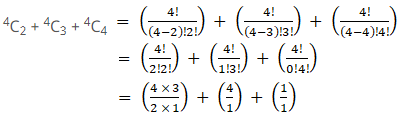= 12/2 + 4 + 1

= 6 + 4 + 1

= 11

∴ The total number of ways of product is 11 ways.

7. From a class of 12 boys and 10 girls, 10 students are to be chosen for the competition, at least including 4 boys and 4 girls. The 2 girls who won the prizes last year should be included. In how many ways can the selection be made?

Solution:

Given:

Total number of boys = 12

Total number of girls = 10

Total number of girls for the competition = 10 + 2 = 12

Number of ways = (no. of ways of selecting 6 boys and 2 girls from remaining 12 boys and 8 girls) + (no. of ways of selecting 5 boys and 3 girls from remaining 12 boys and 8 girls) + (no. of ways of selecting 4 boys and 4 girls from remaining 12 boys and 8 girls)

Since, two girls are already selected,

= (12C6 × 8C2) + (12C5 × 8C3) + (12C4 × 8C4)

By using the formula,

nCr = n!/r!(n – r)!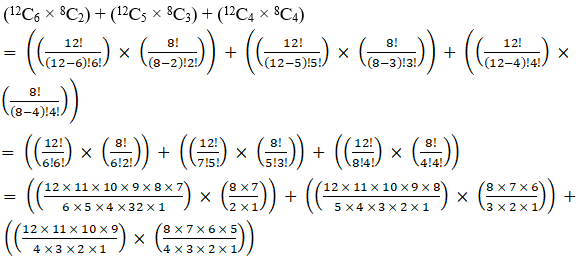= (924 × 28) + (792 × 56) + (495 × 70)

= 25872 + 44352 + 34650

= 104874

∴ The total number of ways of product is 104874 ways.

8. How many different selections of 4 books can be made from 10 different books, if
(i) there is no restriction

(ii) two particular books are always selected

(iii) two particular books are never selected

Solution:

Given:

Total number of books = 10

Total books to be selected = 4

(i) there is no restriction

Number of ways = choosing 4 books out of 10 books

10C4

By using the formula,

nCr = n!/r!(n – r)!

10C4 = 10! / 4! (10 – 4)!

= 10! / (4! 6!)

= [10×9×8×7×6!] / (4! 6!)

= [10×9×8×7] / (4×3×2×1)

= 10×3×7

= 210 ways

(ii) two particular books are always selected

Number of ways = select 2 books out of the remaining 8 books as 2 books are already selected.

= 8C2

By using the formula,

nCr = n!/r!(n – r)!

8C2 = 8! / 2! (8 – 2)!

= 8! / (2! 6!)

= [8×7×6!] / (2! 6!)

= [8×7] / (2×1)

= 4×7

= 28 ways

(iii) two particular books are never selected

Number of ways = select 4 books out of remaining 8 books as 2 books are already removed.

= 8C4

By using the formula,

nCr = n!/r!(n – r)!

8C4 = 8! / 4! (8 – 4)!

= 8! / (4! 4!)

= [8×7×6×5×4!] / (4! 4!)

= [8×7×6×5] / (4×3×2×1)

= 7×2×5

= 70 ways

∴ The required no. of ways are 210, 28, 70.

9. From 4 officers and 8 jawans in how many ways can 6 be chosen (i) to include exactly one officer (ii) to include at least one officer?

Solution:

Given:

Total number of officers = 4

Total number of jawans = 8

Total number of selection to be made is 6

(i) to include exactly one officer

Number of ways = (no. of ways of choosing 1 officer from 4 officers) × (no. of ways of choosing 5 jawans from 8 jawans)

= (4C1) × (8C5)

By using the formula,

nCr = n!/r!(n – r)!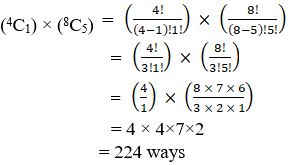(ii) to include at least one officer?

Number of ways = (total no. of ways of choosing 6 persons from all 12 persons) – (no. of ways of choosing 6 persons without any officer)

12C6 – 8C6

By using the formula,

nCr = n!/r!(n – r)!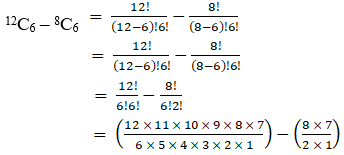= (11×2×3×2×7) – (4×7)

= 924 – 28

= 896 ways

∴ The required no. of ways are 224 and 896.

10. A sports team of 11 students is to be constituted, choosing at least 5 from class XI and at least 5 from class XII. If there are 20 students in each of these classes, in how many ways can the teams be constituted?

Solution:

Given:

Total number of students in XI = 20

Total number of students in XII = 20

Total number of students to be selected in a team = 11 (with atleast 5 from class XI and 5 from class XII)

Number of ways = (No. of ways of selecting 6 students from class XI and 5 students from class XII) + (No. of ways of selecting 5 students from class XI and 6 students from class XII)

= (20C6 × 20C5) + (20C5 × 20C6)

= 2 (20C6 × 20C5) ways

11. A student has to answer 10 questions, choosing at least 4 from each of part A and part B. If there are 6 questions in part A and 7 in part B, in how many ways can the student choose 10 questions?

Solution:

Given:

Total number of questions = 10

Questions in part A = 6

Questions in part B = 7

Number of ways = (No. of ways of answering 4 questions from part A and 6 from part B) + (No. of ways of answering 5 questions from part A and 5 questions from part B) + (No. of ways of answering 6 questions from part A and 4 from part B)

= (6C4 × 7C6) + (6C5 × 7C5) + (6C6 × 7C4)

By using the formula,

nCr = n!/r!(n – r)!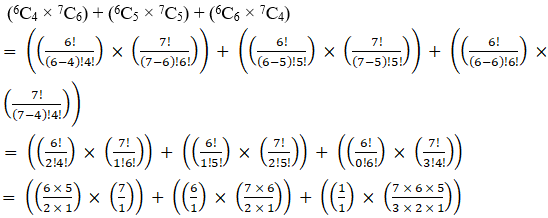= (15×7) + (6×21) + (1×35)

= 105 + 126 + 35

= 266

∴ The total no. of ways of answering 10 questions is 266 ways.

12. In an examination, a student to answer 4 questions out of 5 questions; questions 1 and 2 are however compulsory. Determine the number of ways in which the student can make a choice.

Solution:

Given:

Total number of questions = 5

Total number of questions to be answered = 4

Number of ways = we need to answer 2 questions out of the remaining 3 questions as 1 and 2 are compulsory.

= 3C2

By using the formula,

nCr = n!/r!(n – r)!

3C2 = 3!/2!(3 -2)!

= 3! / (2! 1!)

= [3×2×1] / (2×1)

= 3

∴ The no. of ways answering the questions is 3.

13. A candidate is required to answer 7 questions out of 12 questions which are divided into two groups, each containing 6 questions. He is not permitted to attempt more than 5 questions from either group. In how many ways can he choose the 7 questions?

Solution:

Given:

Total number of questions = 12

Total number of questions to be answered = 7

Number of ways = (No. of ways of answering 5 questions from group 1 and 2 from group 2) + (No. of ways of answering 4 questions from group 1 and 3 from group 2) + (No. of ways of answering 3 questions from group 1 and 4 from group 2) + (No. of ways of answering 2 questions from group 1 and 5 from group 2)

= (6C5 × 6C2) + (6C4 × 6C3) + (6C3 × 6C4) + (6C2 × 6C5)

By using the formula,

nCr = n!/r!(n – r)!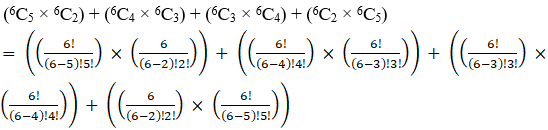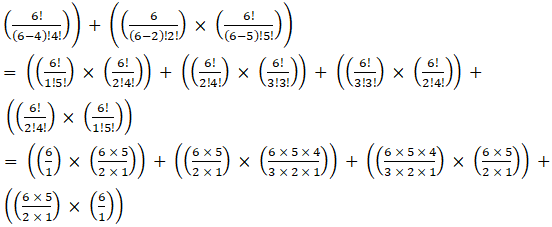= (6×15) + (15×20) + (20×15) + (15×6)

= 90 + 300 + 300 + 90

= 780

∴ The total no. of ways of answering 7 questions is 780 ways.

14. There are 10 points in a plane of which 4 are collinear. How many different straight lines can be drawn by joining these points.

Solution:

Given:

Total number of points = 10

Number of collinear points = 4

Number of lines formed = (total no. of lines formed by all 10 points) – (no. of lines formed by collinear points) + 1

Here, 1 is added because only 1 line can be formed by the four collinear points.

10C2 – 4C2 + 1

By using the formula,

nCr = n!/r!(n – r)!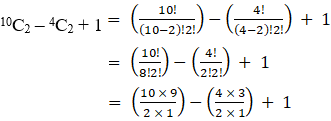= 90/2 – 12/2 + 1

= 45 – 6 + 1

= 40

∴ The total no. of ways of different lines formed are 40.

15. Find the number of diagonals of

(i) a hexagon

(ii) a polygon of 16 sides

Solution:

(i) a hexagon

We know that a hexagon has 6 angular points. By joining those any two angular points we get a line which is either a side or a diagonal.

So number of lines formed = 6C2

By using the formula,

nCr = n!/r!(n – r)!

6C2 = 6!/2!(6-2)!

= 6! / (2! 4!)

= [6×5×4!] / (2! 4!)

= [6×5] / (2×1)

= 3×5

= 15

We know number of sides of hexagon is 6

So, number of diagonals = 15 – 6 = 9

The total no. of diagonals formed is 9.

(ii) a polygon of 16 sides

We know that a polygon of 16 sides has 16 angular points. By joining those any two angular points we get a line which is either a side or a diagonal.

So number of lines formed = 16C2

By using the formula,

nCr = n!/r!(n – r)!

16C2 = 16!/2!(16-2)!

= 16! / (2! 14!)

= [16×15×14!] / (2! 14!)

= [16×15] / (2×1)

= 8×15

= 120

We know number of sides of a polygon is 16

So, number of diagonals = 120 – 16 = 104

The total no. of diagonals formed is 104.

16. How many triangles can be obtained by joining 12 points, five of which are collinear?

Solution:

We know that 3 points are required to draw a triangle and the collinear points will lie on the same line.

Number of triangles formed = (total no. of triangles formed by all 12 points) – (no. of triangles formed by collinear points)

12C3 – 5C3

By using the formula,

nCr = n!/r!(n – r)!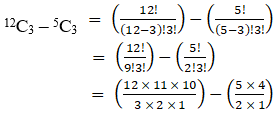= (2×11×10) – (5×2)

= 220 – 10

= 210

∴ The total no. of triangles formed are 210.

EXERCISE 17.3 PAGE NO: 17.23

1. How many different words, each containing 2 vowels and 3 consonants can be formed with 5 vowels and 17 consonants?

Solution:

Given:

Total number of vowels = 5

Total number of consonants = 17

Number of ways = (No. of ways of choosing 2 vowels from 5 vowels) × (No. of ways of choosing 3 consonants from 17 consonants)

= (5C2) × (17C3)

By using the formula,

nCr = n!/r!(n – r)!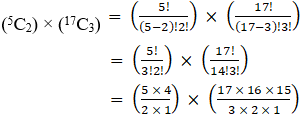= 10 × (17×8×5)

= 10 × 680

= 6800

Now we need to find the no. of words that can be formed by 2 vowels and 3 consonants.

The arrangement is similar to that of arranging n people in n places which are n! Ways to arrange. So, the total no. of words that can be formed is 5!

So, 6800 × 5! = 6800 × (5×4×3×2×1)

= 6800 × 120

= 816000

∴ The no. of words that can be formed containing 2 vowels and 3 consonants are 816000.

2. There are 10 persons named P1, P2, P3 …, P10. Out of 10 persons, 5 persons are to be arranged in a line such that is each arrangement P1 must occur whereas P4 and P5 do not occur. Find the number of such possible arrangements.

Solution:

Given:

Total persons = 10

Number of persons to be selected = 5 from 10 persons (P1, P2, P3 … P10)

It is also told that P1 should be present and P4 and P5 should not be present.

We have to choose 4 persons from remaining 7 persons as P1 is selected and P4 and P5 are already removed.

Number of ways = Selecting 4 persons from remaining 7 persons

7C4

By using the formula,

nCr = n!/r!(n – r)!

7C4 = 7! / 4!(7 – 4)!

= 7! / (4! 3!)

= [7×6×5×4!] / (4! 3!)

= [7×6×5] / (3×2×1)

= 7×5

= 35

Now we need to arrange the chosen 5 people. Since 1 person differs from other.

35 × 5! = 35 × (5×4×3×2×1)

= 4200

∴ The total no. of possible arrangement can be done is 4200.

3. How many words, with or without meaning can be formed from the letters of the word ‘MONDAY’, assuming that no letter is repeated, if
(i) 4 letters are used at a time

(ii) all letters are used at a time

(iii) all letters are used but first letter is a vowel ?

Solution:

Given:

The word ‘MONDAY’

Total letters = 6

(i) 4 letters are used at a time

Number of ways = (No. of ways of choosing 4 letters from MONDAY)

= (6C4)

By using the formula,

nCr = n!/r!(n – r)!

6C4 = 6! / 4!(6 – 4)!

= 6! / (4! 2!)

= [6×5×4!] / (4! 2!)

= [6×5] / (2×1)

= 3×5

= 15

Now we need to find the no. of words that can be formed by 4 letters.

15 × 4! = 15 × (4×3×2×1)

= 15 × 24

= 360

∴ The no. of words that can be formed by 4 letters of MONDAY is 360.

(ii) all letters are used at a time

Total number of letters in the word ‘MONDAY’ is 6

So, the total no. of words that can be formed is 6! = 360

∴ The no. of words that can be formed by 6 letters of MONDAY is 360.

(iii) all letters are used but first letter is a vowel ?

In the word ‘MONDAY’ the vowels are O and A. We need to choose one vowel from these 2 vowels for the first place of the word.

So,

Number of ways = (No. of ways of choosing a vowel from 2 vowels)

= (2C1)

By using the formula,

nCr = n!/r!(n – r)!

2C1 = 2! / 1!(2 – 1)!

= 2! / (1! 1!)

= (2×1)

= 2

Now we need to find the no. of words that can be formed by remaining 5 letters.

2 × 5! = 2 × (5×4×3×2×1)

= 2 × 120

= 240

∴ The no. of words that can be formed by all letters of MONDAY in which the first letter is a vowel is 240.

4. Find the number of permutations of n distinct things taken r together, in which 3 particular things must occur together.

Solution:

Here, it is clear that 3 things are already selected and we need to choose (r – 3) things from the remaining (n – 3) things.

Let us find the no. of ways of choosing (r – 3) things.

Number of ways = (No. of ways of choosing (r – 3) things from remaining (n – 3) things)

n – 3Cr – 3

Now we need to find the no. of permutations than can be formed using 3 things which are together. So, the total no. of words that can be formed is 3!

Now let us assume the together things as a single thing this gives us total (r – 2) things which were present now. So, the total no. of words that can be formed is (r – 2)!

Total number of words formed is:

n – 3Cr – 3 × 3! × (r – 2)!

∴ The no. of permutations that can be formed by r things which are chosen from n things in which 3 things are always together is n – 3Cr – 3 × 3! × (r – 2)!

5. How many words each of 3 vowels and 2 consonants can be formed from the letters of the word INVOLUTE?

Solution:

Given:

The word ‘INVOLUTE’

Total number of letters = 8

Total vowels are = I, O, U, E

Total consonants = N, V, L, T

So number of ways to select 3 vowels is 4C3

And numbre of ways to select 2 consonants is 4C2

Then, number of ways to arrange these 5 letters = 4C3 × 4C2 × 5!

By using the formula,

nCr = n!/r!(n – r)!

4C3 = 4!/3!(4-3)!

= 4!/(3! 1!)

= [4×3!] / 3!

= 4

4C2 = 4!/2!(4-2)!

= 4!/(2! 2!)

= [4×3×2!] / (2! 2!)

= [4×3] / (2×1)

= 2 × 3

= 6

So, by substituting the values we get

4C3 × 4C2 × 5! = 4 × 6 × 5!

= 4 × 6 × (5×4×3×2×1)

= 2880

∴ The no. of words that can be formed containing 3 vowels and 2 consonants chosen from ‘INVOLUTE’ is 2880.

### Also, access exercises of RD Sharma Solutions for Class 11 Maths Chapter 17 – Combinations

Exercise 17.1 Solutions

Exercise 17.2 Solutions

Exercise 17.3 Solutions

## Frequently Asked Questions on RD Sharma Solutions for Class 11 Maths Chapter 17

### Is RD Sharma Solutions for Class 11 Maths Chapter 17 the best source of study material for exam preparation?

Yes, RD Sharma Solutions are the best source of study material for CBSE board exam preparation. The subject experts at BYJU’S outline the concepts in a clear and precise manner, based on the IQ level of students. If you wish to obtain an excellent score, then referring to RD Sharma Solutions is a must. Our solution module utilizes numerous practical examples to explain all the exercise questions in a simple and easily understandable language.

### Where can I download RD Sharma Solutions for Class 11 Maths Chapter 17?

The RD Sharma Solutions for Class 11 Maths Chapter 17 can be referred both in online and offline mode from the BYJU’S website. A team of experts at BYJU’S have prepared the solutions for all the questions available in the RD Sharma textbook for Class 11 in accordance with the latest CBSE guidelines in a simpler steps for students to grasp the concepts easily.

### Where can I get correct RD Sharma Solutions for Class 11 Maths Chapter 17?

RD Sharma textbooks are the best reference materials which provides students and teachers with a wide range of practice questions to answer. Students can find correct and accurate RD Sharma Solutions for Class 11 at BYJU’S website. The solutions are prepared with utmost care to help students understand the concepts easily and score well in their board exams.